Win up to 100% scholarship on Aakash BYJU'S JEE/NEET courses with ABNAT Win up to 100% scholarship on Aakash BYJU'S JEE/NEET courses with ABNAT

# JEE Advanced Question Paper 2022 Physics Paper 2

## JEE Advanced 2022 Physics Question Paper 2 with Solutions

SECTION – 1 (Maximum marks : 24)

∙ This section contains EIGHT (08) questions.

∙ The answer to each question is a SINGLE DIGIT INTEGER ranging from 0 TO 9, BOTH INCLUSIVE.

∙ For each question, enter the correct integer corresponding to the answer using the mouse and the on-screen virtual numeric keypad in the place designated to enter the answer.

∙ Answer to each question will be evaluated according to the following marking scheme:

Full Marks : +3 If ONLY the correct integer is entered;

Zero Marks : 0 If the question is unanswered;

Negative Marks : −1 In all other cases.

1. A particle of mass 1 kg is subjected to a force which depends on the position as

$$\begin{array}{l}\vec F = -k(x\hat i + y\hat j)\,\,{\rm{kg}}\,\,{\rm{m}}{{\rm{s}}^{{\rm{-2}}}}\end{array}$$
with k = 1 kg s–2. At time t = 0, the particle’s position
$$\begin{array}{l}\vec r = \left( {\frac{1}{{\sqrt 2 }}\hat i + \sqrt 2 \hat j} \right)\,\,{\rm{m}}\ \text{and its velocity }\ \vec v = \left( {-\sqrt 2 \hat i + \sqrt 2 \hat j + \frac{2}{\pi }\hat k} \right)\,\,{\rm{m}}{{\rm{s}}^{-1}}.\end{array}$$
Let vx and vy denote the x and the y components of the particle’s velocity, respectively. Ignore gravity. When z = 0.5 m, the value of (xvyyvx) is _______ m2s–1.

Sol. Fx = – x = max.

So

$$\begin{array}{l}{a_x} = \frac{d^2x}{dt^2}=- x\end{array}$$
$$\begin{array}{l} \Rightarrow x = {A_x}\sin \left( {\omega t + {\phi _x}} \right)\end{array}$$

and

$$\begin{array}{l}{v_x} = {A_x}\omega \cos \left( {\omega t + {\phi _x}} \right)\end{array}$$

at t = 0,

$$\begin{array}{l}x = \frac{1}{{\sqrt 2 }}{\rm{m}}\end{array}$$
and
$$\begin{array}{l}v_x=-\sqrt 2\ {\rm{m/s}}\end{array}$$

So

$$\begin{array}{l}\frac{1}{{\sqrt 2 }} = {A_x}\sin {\phi _x}\end{array}$$

and

$$\begin{array}{l} -\sqrt2={A_x}\cos{\phi_x}\end{array}$$
$$\begin{array}{l} \Rightarrow \tan {\phi _x} =-\frac{1}{2}….(1)\end{array}$$

and

$$\begin{array}{l}{A_x} = \sqrt {\frac{5}{2}} \;{\rm{m}}….(2)\end{array}$$

Similarly

Fy = – y = may.

$$\begin{array}{l} \Rightarrow \frac{d^2y}{dt^2}=-y\end{array}$$

So, y = Ay sin(ωt + φy) (ω = 1 rad/s)

and

$$\begin{array}{l}{v_y} = {A_y}\omega \cos \left( {\omega t + {\phi _y}} \right)\end{array}$$

at t = 0, y = √2 m and vy = √2 m/s

So

$$\begin{array}{l}\sqrt 2={A_y}\sin \phi \end{array}$$

and

$$\begin{array}{l}\sqrt 2={A_y}\cos \phi\end{array}$$
$$\begin{array}{l}\Rightarrow \phi=\frac{\pi}{4}\end{array}$$
and Ay = 2 (3 and 4).

So, (xvyyvx)

$$\begin{array}{l}= \sqrt {\frac{5}{2}} \;\sin \;(\omega t + {\phi _x}) \times \;2\;\cos (\omega t + {\phi _y})- \;2\sin (\omega t + {\phi _y}) \times \sqrt {\frac{5}{2}} \;\cos \;(\omega t + {\phi _x})\end{array}$$
$$\begin{array}{l} = \sqrt {\frac{5}{2}}\times 2\;(\sin (\omega t + {\phi _x})\;\cos (\omega t + {\phi _y})-\sin (\omega t + {\phi _y}) \times \,\cos (\omega t + {\phi _x})\end{array}$$
$$\begin{array}{l} = \sqrt {10} \;\sin \;({\phi _x} – \;{\phi _y})\end{array}$$
$$\begin{array}{l} = \sqrt {10} \;(\sin \;{\phi _x}\;\cos {\phi _y} – \;\cos {\phi _x}\;\sin {\phi _y})\end{array}$$
$$\begin{array}{l} = \sqrt {10} \;\left( {\frac{1}{{\sqrt 5 }} \times \frac{1}{{\sqrt 2 }} – \left( { – \frac{2}{{\sqrt 5 }}} \right) \times \frac{1}{{\sqrt 2 }}} \right)\end{array}$$

= 3

2. In a radioactive decay chain reaction,

$$\begin{array}{l}_{90}^{230}{\rm{Th}}\ \text{nucleus decays into}\ _{84}^{214}{\rm{Po}}\ \text{nucleus}.\end{array}$$
The ratio of the number of α to number of β particles emitted in this process is _______.

Sol. Let number of α particles are nα and β particles are nβ so

4nα = 230 – 214

nα = 4

nβ = 84 – (90 – 2nα)

nβ = 2

So

$$\begin{array}{l}\frac{{{n_\alpha }}}{{{n_\beta }}}\; = \;2\end{array}$$

3. Two resistances R1 = X Ω and R2 = 1 Ω are connected to a wire AB of uniform resistivity, as shown in the figure. The radius of the wire varies linearly along its axis from 0.2 mm at A to 1 mm at B. A galvanometer (G) connected to the center of the wire, 50 cm from each end along its axis, shows zero deflection when A and B are connected to a battery. The value of X is _______.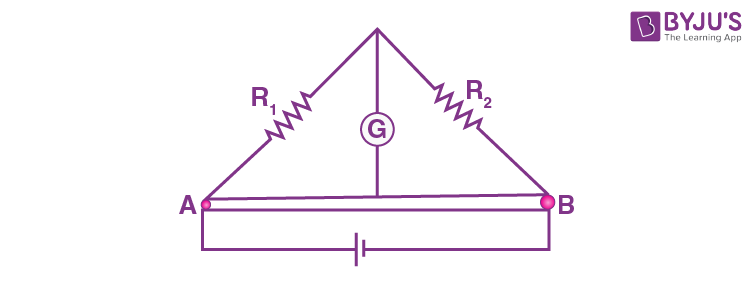Sol. Resistance of frustum shaped conductor shown is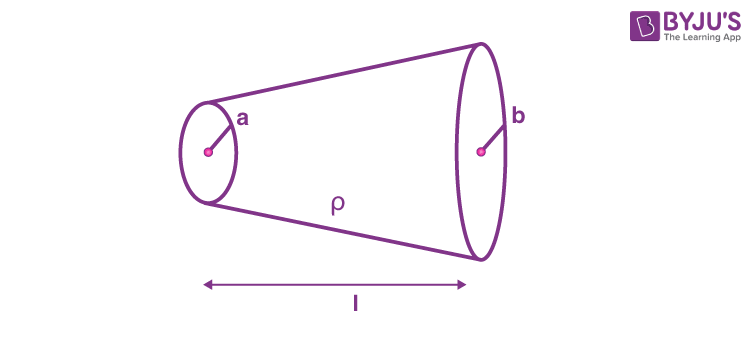$$\begin{array}{l}R = \rho \frac{l}{{\pi ab}}\end{array}$$

For the shown conductor in the diagram.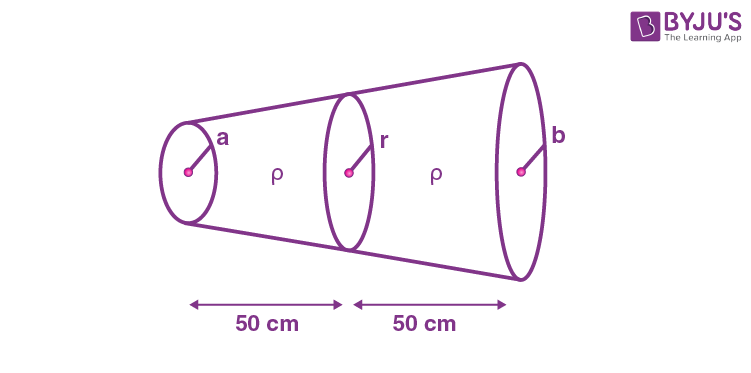$$\begin{array}{l}r = \frac{{a + b}}{2} = \frac{{0.2 + 1}}{2} = 0.6\end{array}$$

thus, the resistance of left half is

$$\begin{array}{l}P=\frac{\rho\times 0.5\times 10^6}{\pi\times 0.2\times 0.6}\end{array}$$

and the resistance of right half is

$$\begin{array}{l}Q=\frac{\rho\times 0.5\times 10^6}{\pi\times 0.6\times 1}\end{array}$$

for Wheatstone to be balanced

$$\begin{array}{l}\frac{{{R_1}}}{P} = \frac{{{R_2}}}{Q}\end{array}$$
$$\begin{array}{l}\frac{{X\ \pi\times 0.2\times 0.6}}{{\rho\times 0.5 \times {{10}^6}}} = \frac{{1\ \pi\times 0.6\times 1}}{{\rho\times 0.5\times {{10}^6}}}\end{array}$$

X = 5

4. In a particular system of units, a physical quantity can be expressed in terms of the electric charge e, electron mass me, Planck’s constant h, and Coulomb’s constant

$$\begin{array}{l}k = \frac{1}{{4\pi {\varepsilon _0}}},\end{array}$$
where ε0 is the permittivity of vacuum. In terms of these physical constants, the dimension of the magnetic field is [B] = [e]α [me]β [h]γ [k]δ. The value of α + β + γ + δ is ______.

Sol. [B] = [e]α [me]β [hγ] [k]δ

[M1T–2I–1] = [IT]α [M]β [ML2T–1]γ [ML3T–4]δ

So, β + γ + δ = 1 …(i)

2γ + 3δ = 0 …(ii)

α – γ – 4δ = –2 …(iii)

α – 2δ = –1 …(iv)

On solving

so, α + β + γ + δ = 4

5. Consider a configuration of n identical units, each consisting of three layers. The first layer is a column of air of height h = ⅓ cm, and the second and third layers are of equal thickness

$$\begin{array}{l}d = \frac{\sqrt3- 1}{2}{\rm{ cm,}}\ \text{and refractive indices}\ {\mu _1} = \sqrt {\frac{3}{2}}\ {\rm{ and }}\ {\mu _2} = \sqrt 3 ,\end{array}$$
respectively. A light source O is placed on the top of the first unit, as shown in the figure. A ray of light from O is incident on the second layer of the first unit at an angle of θ = 60° to the normal. For a specific value of n, the ray of light emerges from the bottom of the configuration at a distance
$$\begin{array}{l}l = \frac{8}{{\sqrt 3 }}{\rm{ cm,}}\end{array}$$
as shown in the figure. The value of n is _______.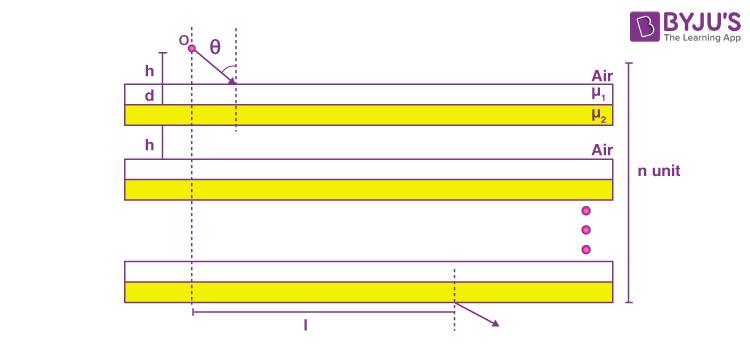Sol.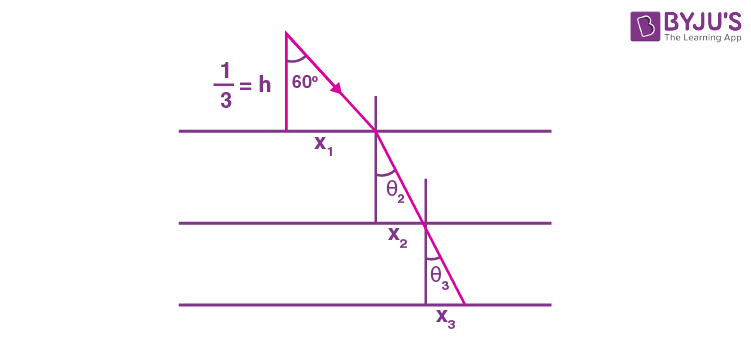$$\begin{array}{l}{x_1}=\frac{1}{3} \times \tan 60^\circ=\frac{1}{{\sqrt 3 }}{\rm{ cm}}\end{array}$$

and,

$$\begin{array}{l}1\times\frac{\sqrt3}{2}=\sqrt{\frac{3}{2}}\times \sin{\theta _2}\end{array}$$

⇒ θ2 = 45°

x2 = d

and,

$$\begin{array}{l}1{\rm{ }} \times {\rm{ }}\frac{{\sqrt 3 }}{2} = \frac{{\sqrt 3 }}{2} \times \sin {\theta _2}\end{array}$$

⇒ θ3 = 30°

$$\begin{array}{l} \Rightarrow {x_3} = \frac{d}{{\sqrt 3 }}\end{array}$$
$$\begin{array}{l} \therefore {x_1} + {x_2} + {x_3} = \frac{1}{{\sqrt 3 }} + \frac{{\left( {\sqrt 3 – 1} \right)}}{2}\left( {1 + \frac{1}{{\sqrt 3 }}} \right)\end{array}$$
$$\begin{array}{l} = \frac{2}{{\sqrt 3 }}{\rm{ cm}}\end{array}$$
$$\begin{array}{l} \therefore n = \frac{l}{{{x_1} + {x_2} + {x_3}}} = \frac{{8/\sqrt 3 }}{{2/\sqrt 3 }} = 4\end{array}$$

6. A charge q is surrounded by a closed surface consisting of an inverted cone of height h and base radius R, and a hemisphere of radius R as shown in the figure. The electric flux through the conical surface is

$$\begin{array}{l}\frac{{nq}}{{6{\varepsilon _0}}}\ \text{(in SI units)}.\end{array}$$
The value of n is _________.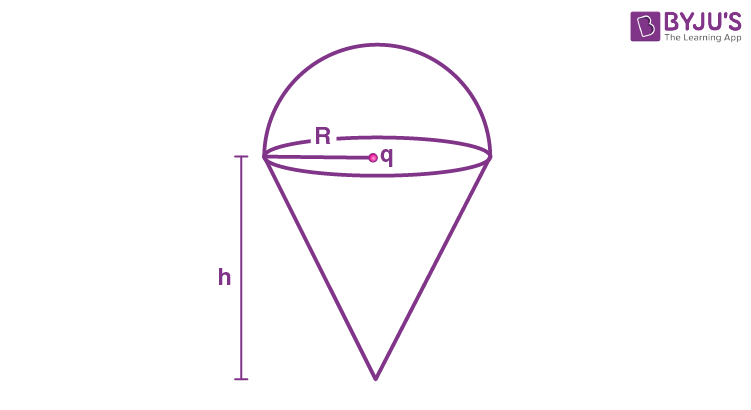Sol.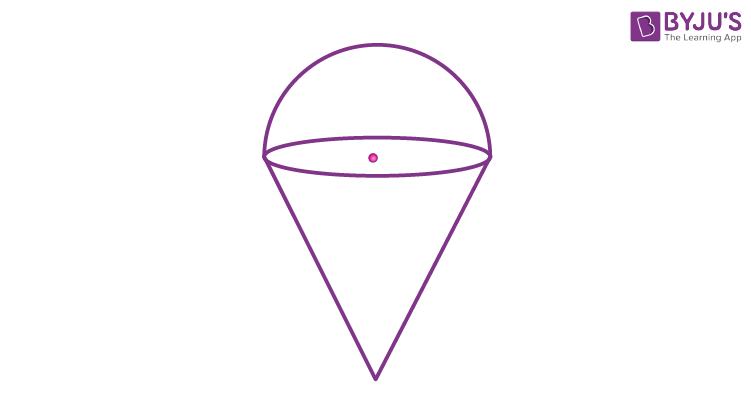φ through cone

$$\begin{array}{l} = \frac{q}{{2{\varepsilon _0}}}\end{array}$$

n = 3

7. On a frictionless horizontal plane, a bob of mass m = 0.1 kg is attached to a spring with natural length l0 = 0.1 m. The spring constant is k1 = 0.009 Nm–1 when the length of the spring l > l0 and is k2 = 0.016 Nm–1 when l < l0. Initially the bob is released from l = 0.15 m. Assume that Hooke’s law remains valid throughout the motion. If the time period of the full oscillation is T = (n π) s, then the integer closest to n is _______.

Sol.$$\begin{array}{l}{\omega _1} = \sqrt {\frac{{{k_1}}}{m}}\ {\rm{ and }}\ {\omega _2} = \sqrt {\frac{{{k_2}}}{m}} \end{array}$$
$$\begin{array}{l} \therefore {\text{Time period}}=\pi \sqrt {\frac{m}{k_1}}+\pi \sqrt {\frac{m}{k_2}}\end{array}$$
$$\begin{array}{l} = \pi \sqrt {\frac{0.1}{0.009}}+ \pi \sqrt{\frac{0.1}{0.016}}\end{array}$$
$$\begin{array}{l} = \frac{\pi }{{0.3}} + \frac{\pi }{{0.4}}\end{array}$$
$$\begin{array}{l} =\pi\times \left( {\frac{4 + 3}{12}} \right)\times 10\end{array}$$
$$\begin{array}{l} = \frac{{70}}{{12}}\pi \end{array}$$

= 5.83π

8. An object and a concave mirror of focal length f = 10 cm both move along the principal axis of the mirror with constant speeds. The object moves with speed V0 = 15 cm s–1 towards the mirror with respect to a laboratory frame. The distance between the object and the mirror at a given moment is denoted by u. When u = 30 cm, the speed of the mirror Vm is such that the image is instantaneously at rest with respect to the laboratory frame, and the object forms a real image. The magnitude of Vm is _____ cm s–1.Sol.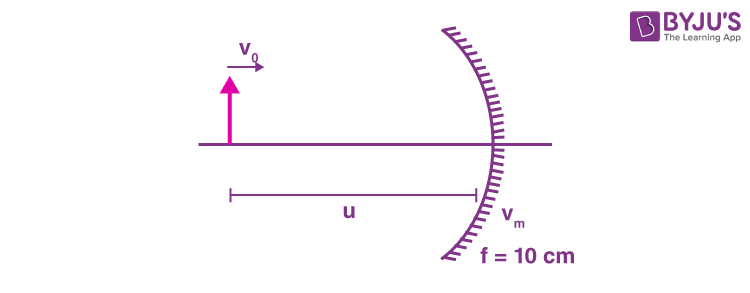$$\begin{array}{l}m = \frac{f}{{u – f}}\end{array}$$
$$\begin{array}{l} = \frac{{10}}{{30 – 10}} = \frac{1}{2}\end{array}$$
$$\begin{array}{l} \therefore \left( {{V_0} – {V_m}} \right) \times {m^2} – {V_m} = 0\end{array}$$
$$\begin{array}{l} \Rightarrow \left( {{V_0} – {V_m}} \right) \times \frac{1}{4}\, = {V_m}\end{array}$$
$$\begin{array}{l} \Rightarrow {V_0} = 5{V_m}\end{array}$$
$$\begin{array}{l} \Rightarrow {V_m} = \frac{{{V_0}}}{5}\end{array}$$
$$\begin{array}{l} = \frac{{15}}{5}\end{array}$$

= 3 cm/s

SECTION – 2 (Maximum marks : 24)

∙ This section contains SIX (06) questions.

∙ Each question has FOUR options (A), (B), (C) and (D). ONE OR MORE THAN ONE of these four option(s) is (are) correct answer(s).

∙ For each question, choose the option(s) corresponding to (all) the correct answer(s).

∙ Answer to each question will be evaluated according to the following marking scheme:

Full Marks : +4 ONLY if (all) the correct option(s) is(are) chosen;

Partial Marks : +3 If all the four options are correct but ONLY three options are chosen;

Partial Marks : + 2 If three or more options are correct but ONLY two options are chosen, both of which are correct;

Partial Marks : +1 If two or more options are correct but ONLY one option is chosen and it is a correct option;

Zero Marks : 0 If none of the options is chosen (i.e. the question is unanswered);

Negative Marks : –2 In all other cases.

9. In the figure, the inner (shaded) region A represents a sphere of radius rA = 1, within which the electrostatic charge density varies with the radial distance r from the center as ρA = kr, where k is positive. In the spherical shell B of outer radius rB, the electrostatic charge density varies as ρB =2k/r. Assume that dimensions are taken care of. All physical quantities are in their SI units.Which of the following statement(s) is/(are) correct?

(A) If

$$\begin{array}{l}{r_B} = \sqrt {\frac{3}{2},} \end{array}$$
then the electric field is zero everywhere outside B.

(B) If

$$\begin{array}{l}{r_B} = \frac{3}{2},\end{array}$$
then the electric potential just outside B is
$$\begin{array}{l}\frac{k}{{{\varepsilon _0}}}.\end{array}$$

(C) If rB = 2, then the total charge of the configuration is 15πk.

(D) lf

$$\begin{array}{l}{r_B} = \frac{5}{2},\end{array}$$
then the magnitude of the electric field just outside B is
$$\begin{array}{l}\frac{{13\pi k}}{{{\varepsilon _0}}}.\end{array}$$

Sol.$$\begin{array}{l}{{\rm{Q}}_{{\rm{Total}}}} = \int\limits_0^{{r_A}} {kr\left( {4\pi {r^2}} \right)dr + \int\limits_{{r_A}}^{{r_B}} {\frac{{2k}}{r}} } \left( {4\pi {r^2}} \right)dr\end{array}$$
$$\begin{array}{l} = \frac{{4\pi k}}{4}r_A^4 + \frac{{8\pi k}}{2}\left( {r_B^2 – r_A^2} \right)\end{array}$$
$$\begin{array}{l} = \pi k + 4\pi k\left( {r_B^2 – r_A^2} \right)\end{array}$$

If

$$\begin{array}{l}{r_B} = \sqrt {\frac{3}{2}} \end{array}$$
$$\begin{array}{l}{Q_{{\rm{Total}}}} = \pi kr_A^4 + 4\pi k\left( {\frac{3}{2} – r_A^2} \right)\end{array}$$
$$\begin{array}{l} = \pi k + 4\pi k\left( {\frac{3}{2} – 1} \right)\end{array}$$
$$\begin{array}{l} = \pi k + 2\pi k = 3\pi k\end{array}$$

If

$$\begin{array}{l}{r_B} = \frac{3}{2}\end{array}$$
$$\begin{array}{l}{Q_{{\rm{Total}}}} = \pi k + 4\pi k\left( {\frac{9}{4} – 1} \right)\end{array}$$
$$\begin{array}{l} = \pi k + 4\pi k\left( {\frac{5}{4}} \right) = 6\pi k\end{array}$$
$$\begin{array}{l}V = \frac{1}{{4\pi {\varepsilon _0}}}\frac{{6\pi k}}{{{r_B}}} = \frac{{3k}}{2}\frac{2}{{3{\varepsilon _0}}} = \frac{k}{{{\varepsilon _0}}}\end{array}$$

(B) is correct

If rB = 2

$$\begin{array}{l}{Q_{{\rm{Total}}}} = \pi k + 4\pi k\left( {4 – 1} \right)\end{array}$$

= 13πk

Option (C) is incorrect

If

$$\begin{array}{l}{r_B} = \frac{5}{2}\end{array}$$
$$\begin{array}{l}{Q_{{\rm{Total}}}} = \pi k + 4\pi k\left( {\frac{{25}}{4} – 1} \right)\end{array}$$
$$\begin{array}{l} = \pi k + \pi k\left( {21} \right)\end{array}$$
$$\begin{array}{l} = 22\pi k\end{array}$$
$$\begin{array}{l}E = \frac{1}{{4\pi {\varepsilon _0}}}\frac{{22\pi k}}{{25}} \times 4\end{array}$$
$$\begin{array}{l} = \frac{{22k}}{{25{\varepsilon _0}}}\end{array}$$

10. In Circuit-1 and Circuit-2 shown in the figures, R1 = 1 Ω, R2 = 2 Ω and R3 = 3 Ω.

P1 and P2 are the power dissipations in Circuit-1 and Circuit-2 when the switches S1 and S2 are in open conditions, respectively.

Q1 and Q2 are the power dissipations in Circuit-1 and Circuit-2 when the switches S1 and S2 are in closed conditions, respectively.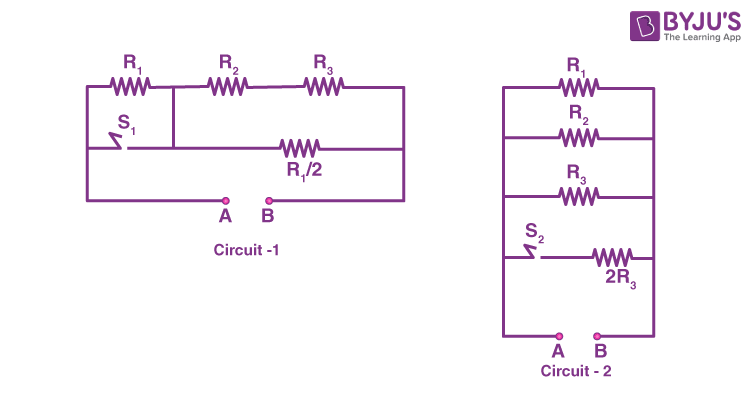Which of the following statement(s) is(are) correct?

(A) When a voltage source of 6 V is connected across A and B in both circuits, P1 < P2

(B) When a constant current source of 2 Amp is connected across A and B in both circuits, P1 > P2

(C) When a voltage source 6 V is connected across A and B in Circuit-1, Q1 > P1

(D) When a constant current source of 2 Amp is connected across A and B in both circuits, Q2 < Q1

Sol.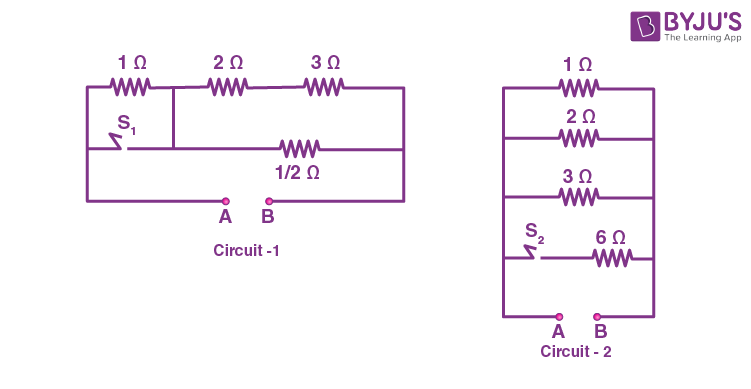When S1 and S2 are open

$$\begin{array}{l}{\left( {{R_{eq}}} \right)_1} = 1 + \frac{{5 \times \frac{1}{2}}}{{5 + \frac{1}{2}}} = 1 + \frac{5}{{11}} = \frac{{16}}{{11}}\end{array}$$
$$\begin{array}{l}{P_1} = \frac{{{V^2}}}{{{R_{eq}}}} = \frac{{{{(6)}^2}}}{{16}} \times 11 = \frac{{36 \times 11}}{{16}} = 24.75{\rm{ W}}\end{array}$$
$$\begin{array}{l}{({R_{eq}})_2} = \frac{6}{{11}}\,\,\Omega \end{array}$$
$$\begin{array}{l}{P_2} = \frac{{{V^2}}}{{{R_{eq}}}} = \frac{{{{(6)}^2}}}{6} \times 11 = \frac{{36 \times 11}}{6} = 66\,\,{\rm{W}}\end{array}$$
$$\begin{array}{l}{P_2} > {P_1}\end{array}$$

Option (A) is correct.

⇒ If 2 A source is used in both the cases.

$$\begin{array}{l}{P_1} = {i^2}{({R_{eq}})_1} = {(2)^2} \times \frac{{16}}{{11}} = \frac{{64}}{{11}} = 5.818\,{\rm{W}}\end{array}$$
$$\begin{array}{l}{P_2} = {i^2}{({R_{eq}})_2} = {(2)^2} \times \frac{6}{{11}} = \frac{{24}}{{11}} = 2.1818\,{\rm{W}}\end{array}$$

P1 > P2

Option (B) is correct

For Q1

$$\begin{array}{l}{R_{eq}} = \frac{5}{{11}}\,\,\Omega \end{array}$$
$$\begin{array}{l}{Q_1} = \frac{{{V^2}}}{{{R_{eq}}}} = \frac{{{{(6)}^2}}}{{\frac{5}{{11}}}} = \frac{{36 \times 11}}{5} = 79.2\,\,{\rm{W}}\end{array}$$

P1 = 24.75 W

Q1 > P1

Option (C) is correct.

For option (D)

$$\begin{array}{l}{Q_1} = {i^2}{R_{eq}} = {(2)^2} \times \frac{5}{{11}} = \frac{{20}}{{11}} = 1.81\,\,{\rm{W}}\end{array}$$
$$\begin{array}{l}{Q_2} = {i^2}{R_{eq}} = {(2)^2} \times \frac{1}{2} = \frac{4}{2} = 2\,\,{\rm{W}}\end{array}$$

Q2 > Q1

Option (D) is incorrect.

11. A bubble has surface tension S. The ideal gas inside the bubble has ratio of specific heats γ = 5/3. The bubble is exposed to the atmosphere and it always retains its spherical shape. When the atmospheric pressure is Pa1, the radius of the bubble is found to be r1 and the temperature of the enclosed gas is T1. When the atmospheric pressure is Pa2, the radius of the bubble and the temperature of the enclosed gas are r2 and T2, respectively.

Which of the following statement(s) is(are) correct?

(A) If the surface of the bubble is a perfect heat insulator, then

$$\begin{array}{l}{\left( {\frac{{{r_1}}}{{{r_2}}}} \right)^5} = \frac{{{P_{a2}} + \frac{{2S}}{{{r_2}}}}}{{{P_{a1}} + \frac{{2S}}{{{r_1}}}}}\end{array}$$

(B) If the surface of the bubble is a perfect heat insulator, then the total internal energy of the bubble including its surface energy does not change with the external atmospheric pressure.

(C) If the surface of the bubble is a perfect heat conductor and the change in atmospheric temperature is negligible, then

$$\begin{array}{l}{\left( {\frac{{{r_1}}}{{{r_2}}}} \right)^3} = \frac{{{P_{a2}} + \frac{{4S}}{{{r_2}}}}}{{{P_{a1}} + \frac{{4S}}{{{r_1}}}}}\end{array}$$

(D) If the surface of the bubble is a perfect heat insulator, then

$$\begin{array}{l}{\left( {\frac{{{T_2}}}{{{T_1}}}} \right)^{\frac{5}{2}}} = \frac{{{P_{a2}} + \frac{{4S}}{{{r_2}}}}}{{{P_{a1}} + \frac{{4S}}{{{r_1}}}}}\end{array}$$

Sol. S : Surface tension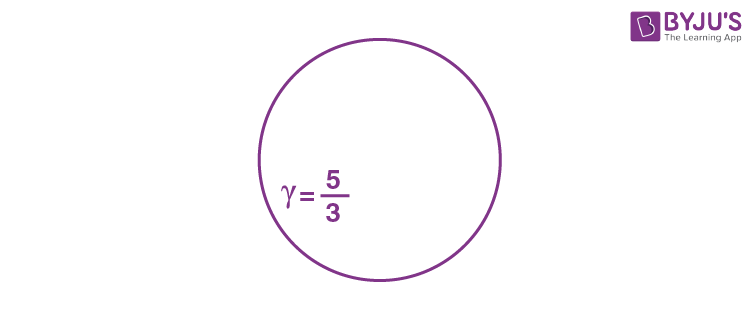When,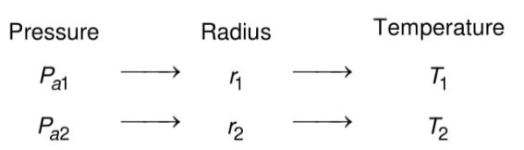$$\begin{array}{l}{P_1}{V_1}^\gamma={P_2}{V_2}^\gamma \end{array}$$
$$\begin{array}{l}\left( {{P_{a1}} + \frac{{4T}}{{{r_1}}}} \right){\left( {\frac{4}{3}\pi r_1^3} \right)^{\frac{5}{3}}} = \left( {{P_{a2}} + \frac{{4T}}{{{r_2}}}} \right){\left( {\frac{4}{3}\pi r_2^3} \right)^{\frac{5}{3}}}\end{array}$$
$$\begin{array}{l}{\left({\frac{{r_1}}{{r_2}}} \right)^5}=\frac{{\left({{P_{a2}} + \frac{4T}{r_2}} \right)}}{{\left({{P_{a1}}+\frac{{4T}}{{r_1}}} \right)}}\end{array}$$

___________________

$$\begin{array}{l}{T_1}{V_1}^{\gamma -1} = {T_2}{V_2}^{\gamma -1}\end{array}$$
$$\begin{array}{l}\frac{{{T_2}}}{{{T_1}}} = {\left( {\frac{{{V_1}}}{{{V_2}}}} \right)^{\gamma -1}} = {\left( {\frac{{{r_1}}}{{{r_2}}}} \right)^{3\left( {\frac{2}{3}} \right)}}\end{array}$$
$$\begin{array}{l}\left ( \frac{T_2}{T_1} \right )=\left ( \frac{P_{a2}+\frac{4T}{r_2}}{P_{a1}+\frac{4T}{r_1}} \right )^\frac{2}{5}\end{array}$$

For option (B) Total internal energy + surface energy will not be same as word done by gas will be there.

Option (B) is incorrect.

For option (C)

$$\begin{array}{l}{P_1}{V_1} = {P_2}{V_2}\end{array}$$
$$\begin{array}{l}\left( {{P_{a1}} + \frac{{4T}}{{{r_1}}}} \right)\left( {\frac{4}{3}\pi r_1^3} \right) = \left( {{P_{a2}} + \frac{{4T}}{{{r_2}}}} \right)\left( {\frac{4}{3}\pi r_2^3} \right)\end{array}$$
$$\begin{array}{l}\left ( \frac{r_1}{r_2} \right )^3=\left ( \frac{P_{a2}+\frac{4T}{r_2}}{P_{a1}+\frac{4T}{r_1}} \right )\end{array}$$

Option (C) is correct

12. A disk of radius R with uniform positive charge density σ is placed on the xy plane with its center at the origin. The Coulomb potential along the z-axis is

$$\begin{array}{l}V(z) = \frac{\sigma }{{2{ \in _0}}}\left( {\sqrt {{R^2} + {z^2}} -z} \right)\end{array}$$

A particle of positive charge q is placed initially at rest at a point on the z-axis with z = z0 and z0 > 0. In addition to the Coulomb force, the particle experiences a vertical force

$$\begin{array}{l}\overrightarrow F=-c\ \hat k\ \text{with}\ c > 0.\ \text{Let}\ \beta=\frac{{2c{\in _0}}}{{q\sigma }}.\end{array}$$
Which of the following statement(s) is(are) correct?

(A) For

$$\begin{array}{l}\beta=\frac{1}{4}\ {\rm{ and}}\ {z_0} = \frac{{25}}{7}R,\end{array}$$
the particle reaches the origin.

(B) For

$$\begin{array}{l}\beta=\frac{1}{4}\ {\rm{ and}}\ {z_0} = \frac{3}{7}R,\end{array}$$
the particle reaches the origin.

(C) For

$$\begin{array}{l}\beta=\frac{1}{4}\ {\rm{ and}}\ {z_0} = \frac{R}{{\sqrt 3 }},\end{array}$$
the particle returns back to z = z0

(D) For β > 1 and z0 > 0, the particle always reaches the origin.

Sol.

$$\begin{array}{l}V(z) = \frac{\sigma }{{2{ \in _0}}}\left( {\sqrt {{R^2} + {z^2}} -z} \right)\end{array}$$

Uz = cz

$$\begin{array}{l}\Rightarrow U{(z)_{net}}=\frac{{\sigma q}}{{2{ \in _0}}}\left( {\sqrt {{R^2} + {z^2}} -z} \right) + cz\end{array}$$
$$\begin{array}{l}=c\left[ {\frac{{\sigma q}}{{2c{ \in _0}}}\left( {\sqrt {{R^2} + {z^2}} -z} \right) + z} \right]\end{array}$$
$$\begin{array}{l} =c\left[{4\sqrt{{R^2} + {z^2}} -3z} \right]{\rm{ at }}\beta=\frac{1}{4}\end{array}$$

At z = 0,

$$\begin{array}{l}\beta = \frac{1}{4}\end{array}$$
$$\begin{array}{l}U{(z)_{net}} = c\left[ {4R} \right] = 4Rc….(i)\end{array}$$

At z = z0 = 25/7 R, β = ¼

$$\begin{array}{l}U{(z)_{net}} = c\left[ {4 \times \frac{{26R}}{7}-3 \times \frac{{25R}}{7}} \right] = \frac{{29}}{7}Rc….(ii)\end{array}$$

At z = z0 = 3/7 R, β = ¼

$$\begin{array}{l}U{(z)_{net}} = c\left[ {4 \times \frac{{\sqrt {58} }}{7}R-\frac{{9R}}{7}} \right] \approx 3Rc….(iii)\end{array}$$
$$\begin{array}{l}\text{At}\ z =\frac{R}{{\sqrt 3 }}\ \beta=\frac{1}{4}\end{array}$$
$$\begin{array}{l}U{(z)_{net}} = c\left[ {\frac{{8R}}{{\sqrt 3 }}-\frac{{3R}}{{\sqrt 3 }}} \right] \approx 2.887\,Rc\end{array}$$

⇒ In option (A) particle reaches at origin with positive kinetic energy

$$\begin{array}{l}\frac{{dU(z)}}{{dz}} = 0\,\,{\rm{at}}\,\,z = \frac{{3R}}{{\sqrt 7 }}\end{array}$$
$$\begin{array}{l}{\rm{at}}\,\,\,\beta \,{\rm{ = }}\frac{1}{4}{\rm{ and}}\,\,z = \frac{{3R}}{{\sqrt 7 }}\end{array}$$
$$\begin{array}{l}U{(z)_{{\rm{net}}}} = \sqrt 7 Rc = 2.645\end{array}$$

⇒ In option B at U(z)net

$$\begin{array}{l} \approx 3Rc\end{array}$$

⇒ The kinetic energy at origin will become negative

$$\begin{array}{l}{\rm{at}}\,\,z = \frac{R}{{\sqrt 3 }}\end{array}$$

⇒ In option (C),

$$\begin{array}{l}U{(z)_{net}}\,{\rm{at}}\,z = \frac{R}{{\sqrt 3 }} < U(z){\,_{net}}\end{array}$$
at z = 0,

And

$$\begin{array}{l}\begin{array}{l}U{(z)_{net}}\,{\rm{at}}\,z = \frac{R}{{\sqrt 3 }} > U(z){\,_{net}}\,\,{\rm{at}}\,z = \frac{{3R}}{{\sqrt 7 }}\\{\rm{ }}\end{array}\end{array}$$

⇒ Particle will return back to z0.

In option (D) (β > 1 and z0 > 0)

U(z)net will keep on increasing with z

⇒ Particle always reaches the origin.

13. A double slit setup is shown in the figure. One of the slits is in medium 2 of refractive index n2. The other slit is at the interface of this medium with another medium 1 of refractive index n1 (≠ n2). The line joining the slits is perpendicular to the interface and the distance between the slits is d. The slit widths are much smaller than d. A monochromatic parallel beam of light is incident on the slits from medium 1. A detector is placed in medium 2 at a large distance from the slits, and at an angle θ from the line joining them, so that θ equals the angle of refraction of the beam. Consider two approximately parallel rays from the slits received by the detector.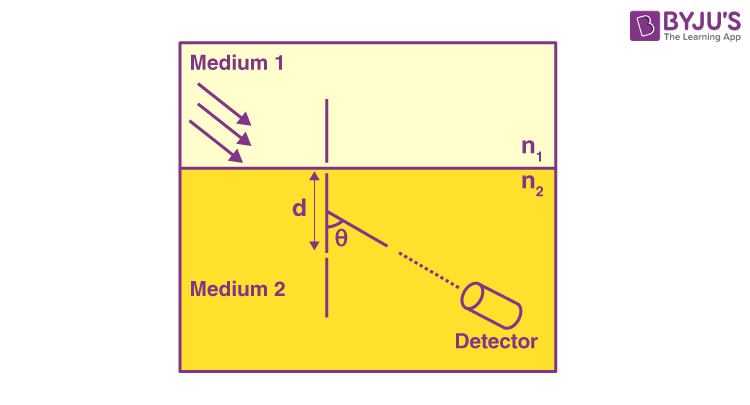Which of the following statement(s) is(are) correct?

(A) The phase difference between the two rays is independent of d.

(B) The two rays interfere constructively at the detector.

(C) The phase difference between the two rays depends on n1 but is independent of n2.

(D) The phase difference between the two rays vanishes only for certain values of d and the angle of incidence of the beam, with q being the corresponding angle of refraction.

Sol.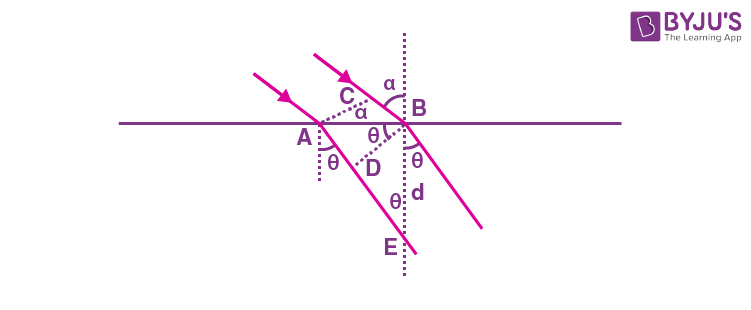AB = (d)(tanθ)

and BC = AB sinα = (d) (tanθ)(sinα)

⇒ Path difference (in vaccum)

= n1(AB) sinα – n2(AB sinθ)

= AB (n1 sinα – n2 sinθ) = 0

⇒ (A), (B) are correct

(C), (D) are incorrect.

14. In the given PV diagram, a monoatomic gas (γ = 5/3) is first compressed adiabatically from state A state B. Then it expands isothermally from state B to state C. [Given :

$$\begin{array}{l}{\left( {\frac{1}{3}} \right)^{0.6}}\; \simeq \;0.5,\;{\rm{In}}\;2\; \simeq \;0.7\end{array}$$
].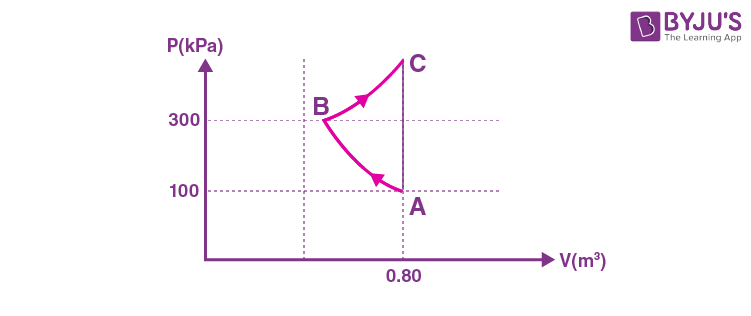Which of the following statement(s) is(are) correct?

(A) The magnitude of the total work done in the process ABC is 144 kJ.

(B) The magnitude of the work done in the process BC is 84 kJ.

(C) The magnitude of the work done in the process AB is 60 kJ.

(D) The magnitude of the work done in the process CA is zero.

Sol.Pvγ = c

$$\begin{array}{l} \Rightarrow 100{(0.8)^{5/3}}\; = \;300{(v)^{5/3}}\end{array}$$
$$\begin{array}{l} \Rightarrow {V_B} = \;\frac{{0.8}}{{{3^{3/5}}}}\end{array}$$
$$\begin{array}{l} \Rightarrow W_{AB}=\frac{{P_AV_A-P_BV_B}}{{\frac{5}{3} – 1}}=\frac{80 – 300 \times \frac{{0.8}}{{{3^{3/5}}}}}{{2/3}}\ {\rm{kJ}}\end{array}$$
$$\begin{array}{l} = \frac{{80 – 240(0.5)}}{{2/3}}\;{\rm{kJ}}\end{array}$$

= – 60 kJ

⇒ (C) is correct

CA is isochoric ⇒ (D) is correct

BC : WBC

$$\begin{array}{l} = \;nRT\;\ln \;\frac{{{V_2}}}{{{V_1}}}\; = \;PV\;\ln \;\frac{{{V_2}}}{{{V_1}}}\end{array}$$
$$\begin{array}{l} = 300 \times 0.8 \times 0.5\ln \left( {\frac{{0.8}}{{\frac{{0.8}}{{{3^{3/5}}}}}}} \right)\end{array}$$
$$\begin{array}{l}=\frac{240}{3^{3/5}}\ln(3^{3/5})\end{array}$$
$$\begin{array}{l}=120\times \frac{3}{5}\ln 3\ kJ\end{array}$$

= 79 kJ

⇒ (A) & (B) are not correct.

SECTION – 3 (Maximum marks : 12)

∙ This section contains FOUR (04) questions.

∙ Each question has FOUR options (A), (B), (C) and (D). ONLY ONE of these four options is the correct answer.

∙ For each question, choose the option corresponding to the correct answer.

∙ Answer to each question will be evaluated according to the following marking scheme:

Full Marks : +3 If ONLY the correct option is chosen;

Zero Marks : 0 If none of the options is chosen (i.e. the question is unanswered);

Negative Marks : −1 In all other cases.

15. A flat surface of a thin uniform disk A of radius R is glued to a horizontal table. Another thin uniform disk B of mass M and with the same radius R rolls without slipping on the circumference of A, as shown in the figure. A flat surface of B also lies on the plane of the table. The center of mass of B has fixed angular speed ω about the vertical axis passing through the center of A. The angular momentum of B is nMωR2 with respect to the center of A. Which of the following is the value of n?(A) 2

(B) 5

(C) 7/2

(D) 9/2

Sol. Angular momentum of B with respect to center of A

$$\begin{array}{l}\vec L\; = \;{\vec L_{{\rm{CM}}}}\; + \;{\vec L_{\,{\rm{Body}}\;{\rm{about}}\;{\rm{CM}}}}\end{array}$$
$$\begin{array}{l} = \;M{(2R)^2}\omega \hat k\; + \;\frac{{M{R^2}}}{2}({\omega _{{\rm{body}}}})\,\hat k\end{array}$$
$$\begin{array}{l} = \;M{(2R)^2}\omega \,\hat k\; + \;\frac{{M{R^2}}}{2}\,(2\omega )\,\hat k\end{array}$$
$$\begin{array}{l} = \;5M{R^2}\omega \hat k\end{array}$$

Comparing the magnitude with nMωR2

n = 5

16. When light of a given wavelength is incident on a metallic surface, the minimum potential needed to stop the emitted photoelectrons is 6.0 V. This potential drops to 0.6 V if another source with wavelength four times that of the first one and intensity half of the first one is used. What are the wavelength of the first source and the work function of the metal, respectively? [Take hc/e = 1.24 × 10–6 J m C–1.]

(A) 1.72 x 10–7 m, 1.20 eV

(B) 1.72 x 10–7 m, 5.60 eV

(C) 3.78 x 10–7 m, 5.60 eV

(D) 3.78 x 10–7 m, 1.20 eV

Sol.

$$\begin{array}{l}h\nu-\phi=6\ eV\end{array}$$
$$\begin{array}{l}\frac{{hc}}{\lambda } -\phi= 6\;{\rm{eV}}….(i)\end{array}$$
$$\begin{array}{l}\frac{{hc}}{{4\lambda }}-\phi= 0.6\ {\rm{eV}}\end{array}$$
$$\begin{array}{l}\frac{{3hc}}{{4\lambda }}= 5.4\ {\rm{eV}}\end{array}$$
$$\begin{array}{l} \therefore \lambda=\frac{{3hc}}{{4 \times 5.4\ {\rm{eV}}}}=\frac{{3 \times 1.24 \times {{10}^{-6}}}}{{4 \times 5.4}}\end{array}$$

= 1.72 × 10–7 m

⇒ from equation (i)

$$\begin{array}{l}\frac{{hc}}{{1.72 \times {{10}^{-7}}}} \times \frac{1}{{1.6 \times {{10}^{-19}}}}-\phi=6\ {\rm{eV}}\end{array}$$
$$\begin{array}{l}\frac{{2 \times {{10}^{ – 25}}}}{{2.75 \times {{10}^{ – 26}}}}-\phi=6\end{array}$$
$$\begin{array}{l} \Rightarrow \phi=\left( {7.27 – 6} \right) \cong 1.2\ {\rm{eV}}\end{array}$$

17. Area of the cross-section of a wire is measured using a screw gauge. The pitch of the main scale is 0.5 mm. The circular scale has 100 divisions and for one full rotation of the circular scale, the main scale shifts by two divisions. The measured readings are listed below.

Two arms of gauge touching each other without wire 0 division 4 divisions
Attempt-1: With wire 4 divisions 20 divisions
Attempt-2: With wire 4 divisions 16 divisions

What are the diameter and cross-sectional area of the wire measured using the screw gauge?

(A) 2.22 ± 0.02 mm, π (1.23 ± 0.02) mm2

(B) 2.22 ± 0.01 mm, π (1.23 ± 0.01) mm2

(C) 2.14 ± 0.02 mm, π (1.14 ± 0.02) mm2

(D) 2.14 ± 0.01 mm, π (1.14 ± 0.01) mm2

Answer (C*) (Bonus declared by JEE)

MSR = 4 × 0.5 = 2 mm

$$\begin{array}{l}{\rm{CSR}} = \frac{1}{100}\times 20 = 0.20\ mm\end{array}$$

Zero error

$$\begin{array}{l} = \frac{1}{{100}}\times 4 = 0.04\;{\rm{mm}}\end{array}$$

R1 = MSR + CSR – (Zero error)

= (2 + 0.20 – 0.04) mm

= 2.16 mm

MSR = 2 mm

CSR = 0.16 mm

Zero error = 0.04 mm

R2 = (2 + 0.16 – 0.04) mm = 2.12 mm

$$\begin{array}{l} = \frac{{{{\rm{R}}_1} + {{\rm{R}}_2}}}{2}\end{array}$$
= 2.14 mm = Rm (say)

Average mean error

$$\begin{array}{l} = \frac{{{\rm{|}}{R_m} – {R_1}{\rm{|}}\,\,{\rm{ + }}\,\,{\rm{|}}{R_m} – {R_2}{\rm{|}}}}{2}\end{array}$$
= 0.02 mm

⇒ Diameter = (2.14 ± 0.02) mm = d (say)

Area

$$\begin{array}{l} = \frac{{\pi \;{d^2}}}{4}\end{array}$$
$$\begin{array}{l} \Rightarrow dA= \frac{{2\pi d}}{4}(\Delta d)\end{array}$$
$$\begin{array}{l} \Rightarrow \Delta {\rm{Area}} \cong \pi \,(0.02)\end{array}$$
$$\begin{array}{l} \Rightarrow {\rm{Area}} = \frac{{\pi {d^2}}}{4} \pm \Delta {\rm{Area}} = \pi \;\left( {1.14 \pm 0.02} \right)\;\,{\rm{mm}}\end{array}$$

18. Which one of the following options represents the magnetic field

$$\begin{array}{l}\vec B \end{array}$$
at O due to the current flowing in the given wire segments lying on the xy plane?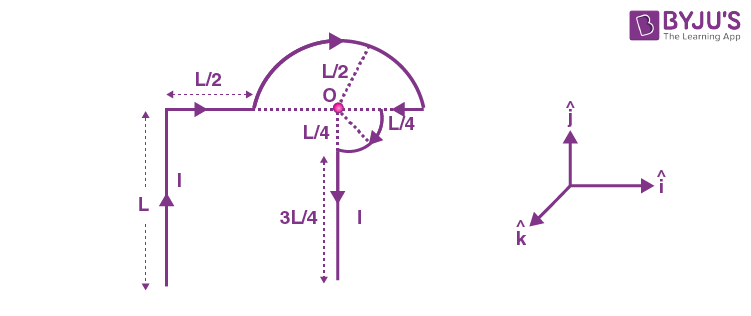(A)

$$\begin{array}{l}\overrightarrow B = \frac{{ – {\mu _0}I}}{L}\;\left( {\frac{3}{2} + \frac{1}{{4\sqrt 2 \;\pi }}} \right)\hat k\end{array}$$

(B)

$$\begin{array}{l}\overrightarrow B=\frac{{-{\mu _0}I}}{L}\left( {\frac{3}{2} + \frac{1}{{2\sqrt 2 \ \pi }}} \right)\hat k\end{array}$$

(C)

$$\begin{array}{l}\overrightarrow B=\frac{{ – {\mu _0}I}}{L}\;\left( {1 + \frac{1}{{4\sqrt 2 \;\pi }}} \right)\hat k\end{array}$$
(D)
$$\begin{array}{l}\overrightarrow B=\frac{{ – {\mu _0}I}}{L}\;\left( {1 + \frac{1}{{4\pi }}} \right)\hat k\end{array}$$

Sol.Bnet = Bsemicircle + Bquarter + Bstraight

$$\begin{array}{l} =\frac{\mu _0I}{4\left(\frac{L}{2}\right)}+\frac{\mu _0I}{8\times\left(\frac{L}{4}\right)}+\frac{\mu _0I}{4\pi\times L}\left(\frac{1}{\sqrt{2}}\right)\left(-\hat{k}\right)\end{array}$$
$$\begin{array}{l} = \left( {\frac{{{\mu _0}I}}{{2L}} + \frac{{{\mu _0}I}}{{2L}} + \frac{{{\mu _0}I}}{{4\sqrt 2 \pi L}}} \right)\;\left( { – \hat k} \right)\end{array}$$
$$\begin{array}{l} = \frac{{{\mu _0}I}}{L}\left( {1 + \frac{1}{{4\sqrt 2 \;\pi }}} \right)\;\left( { – \hat k} \right)\end{array}$$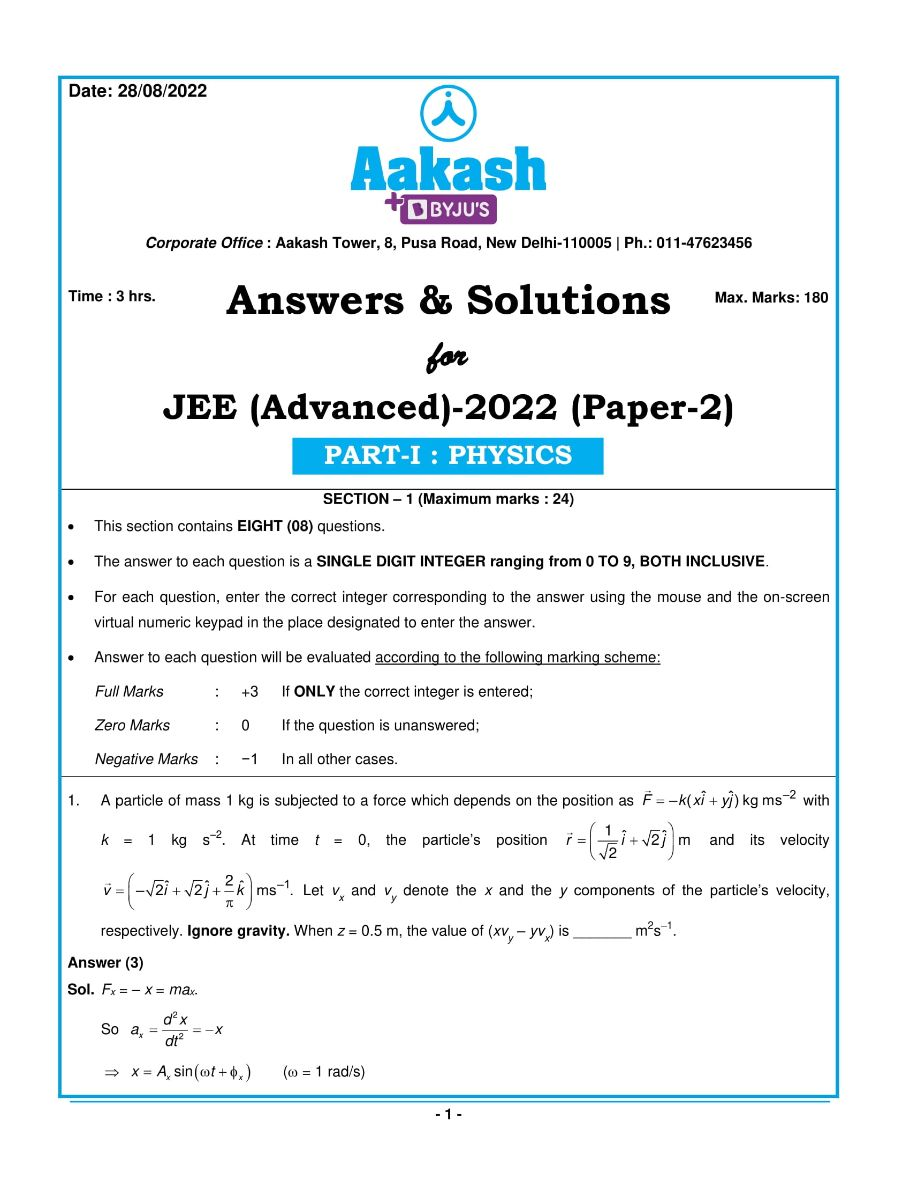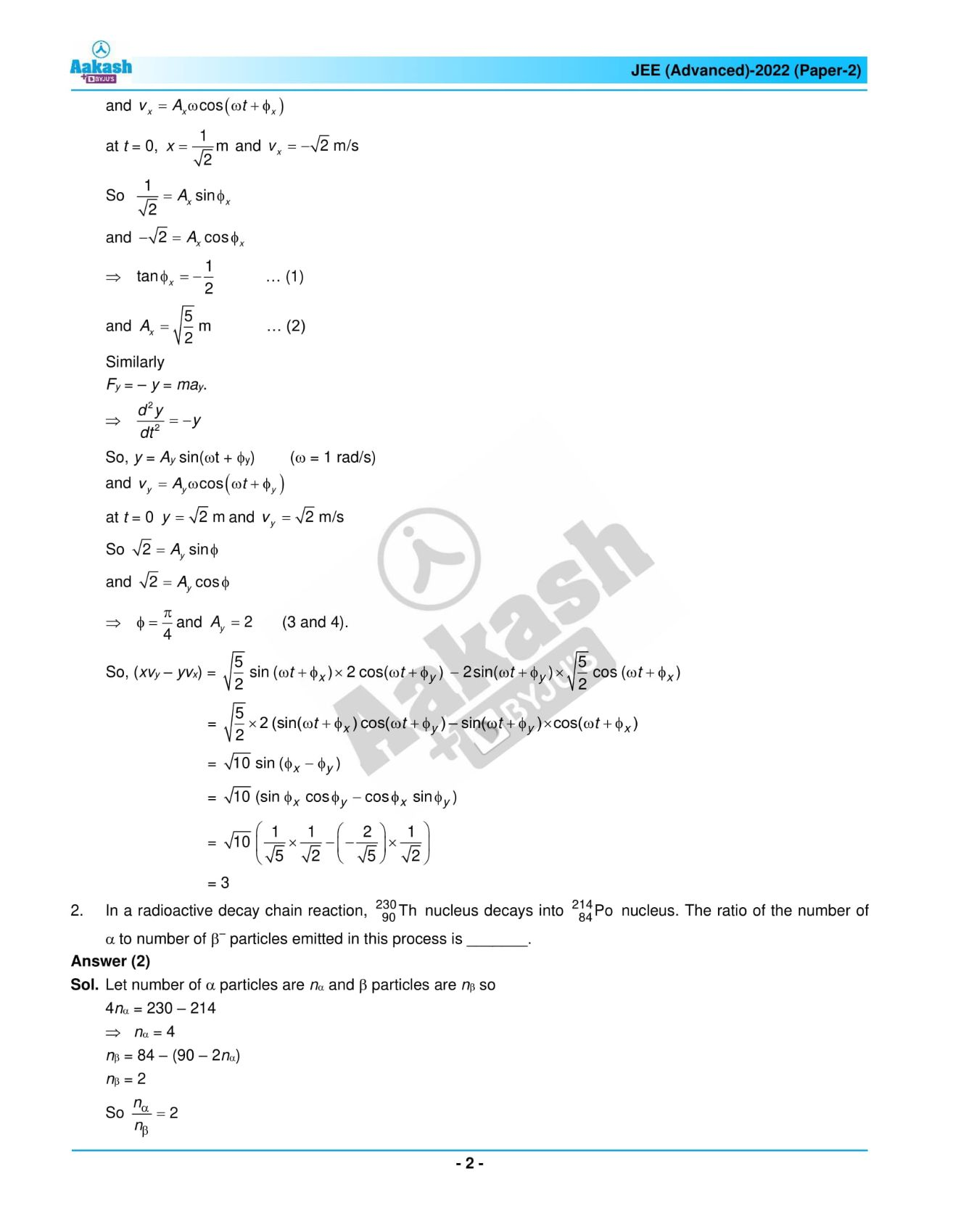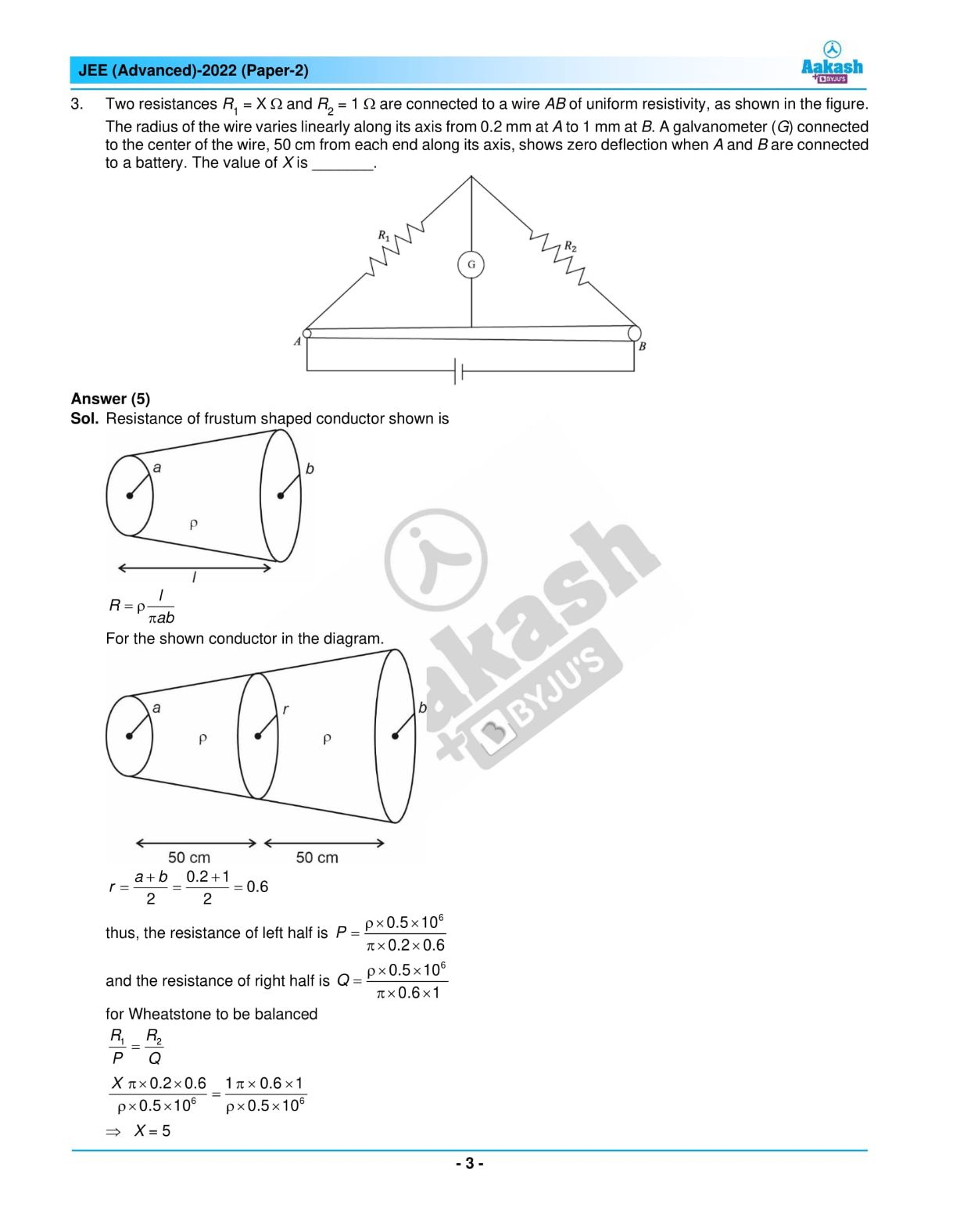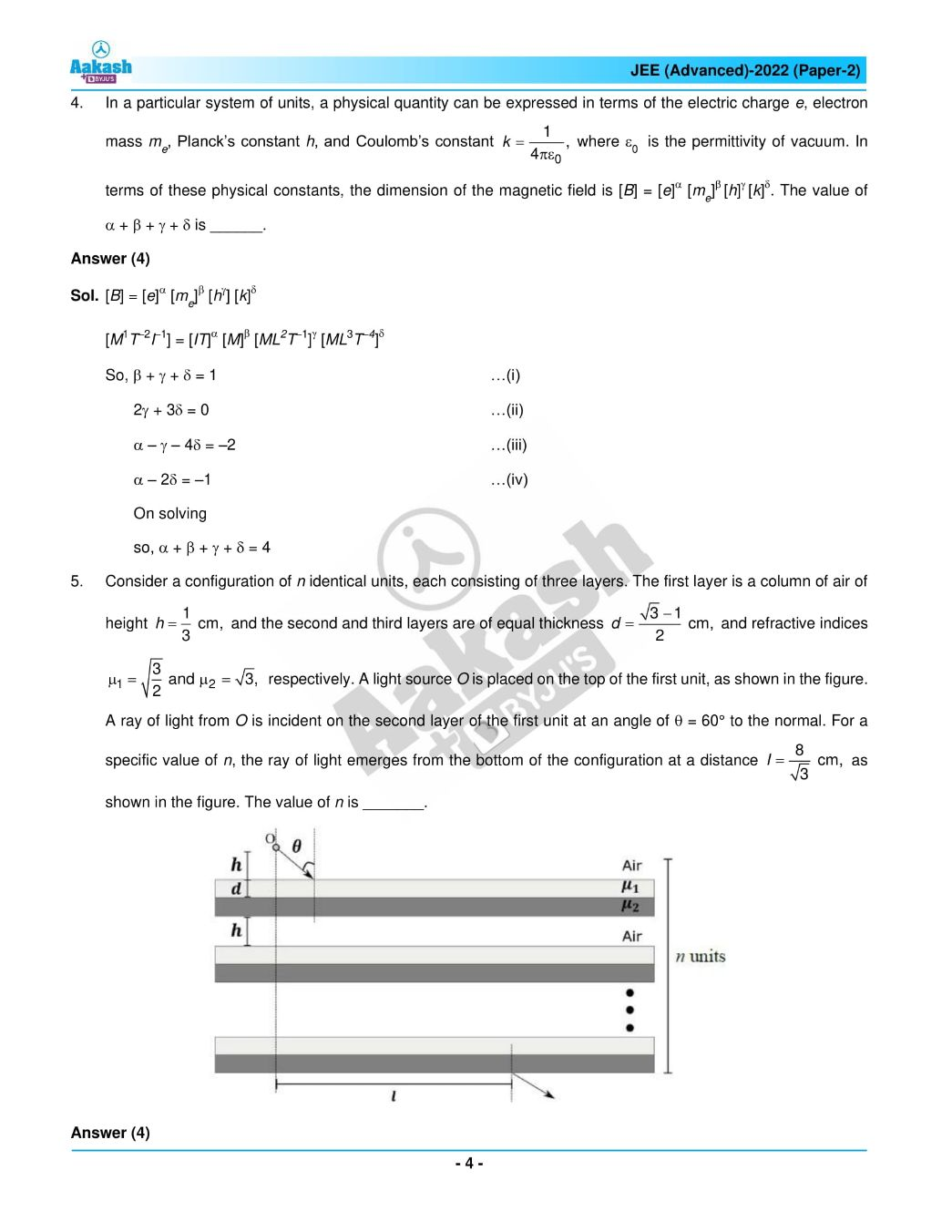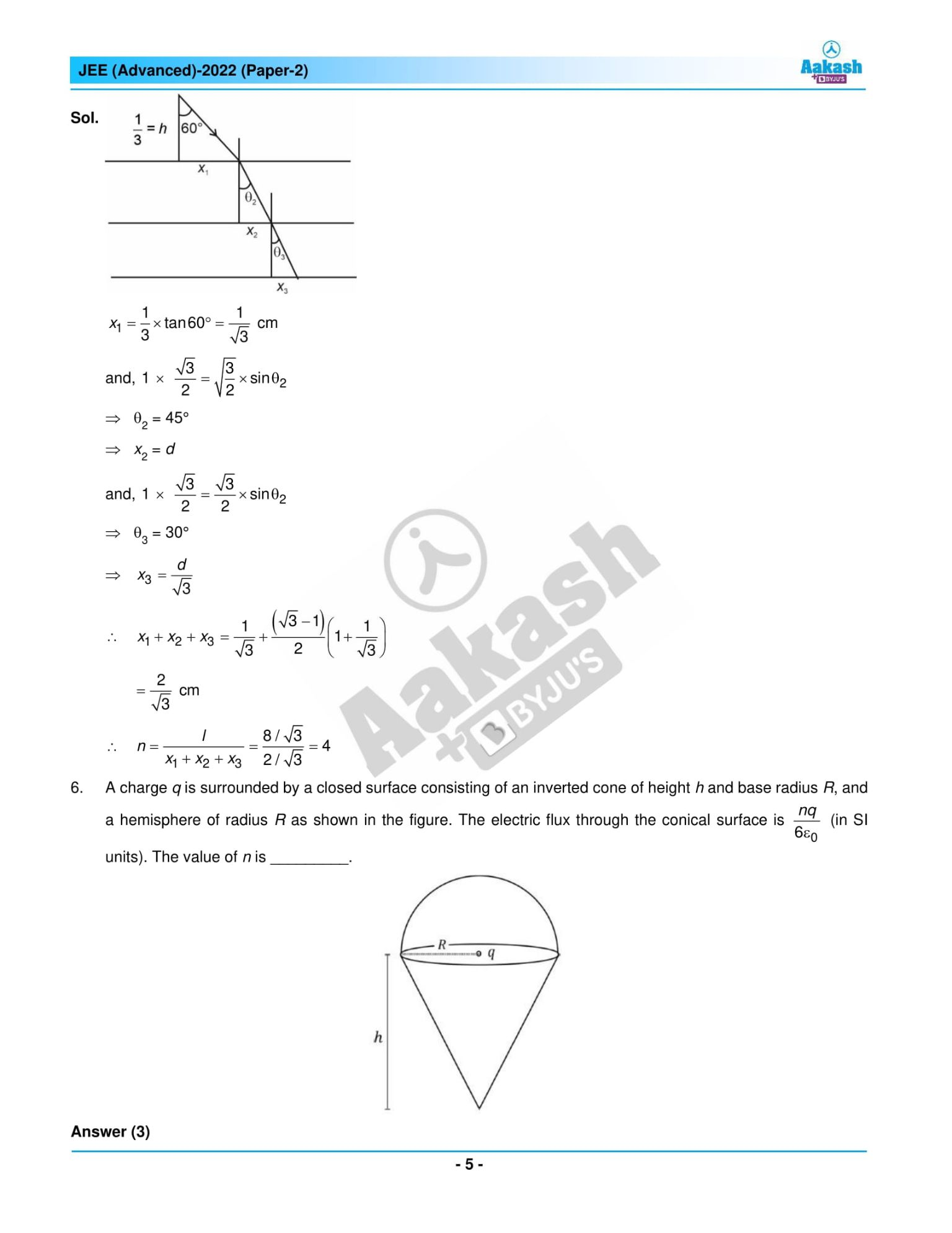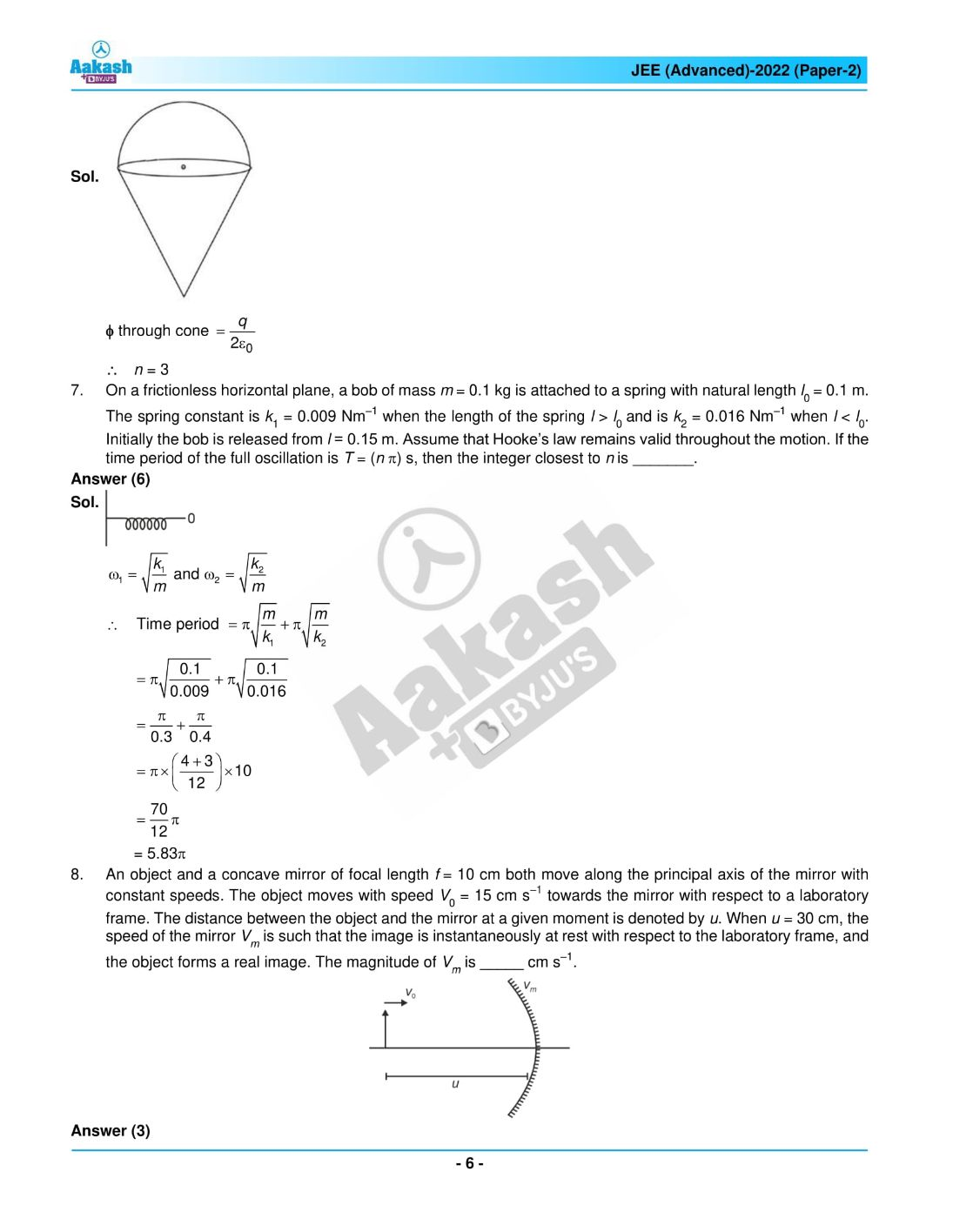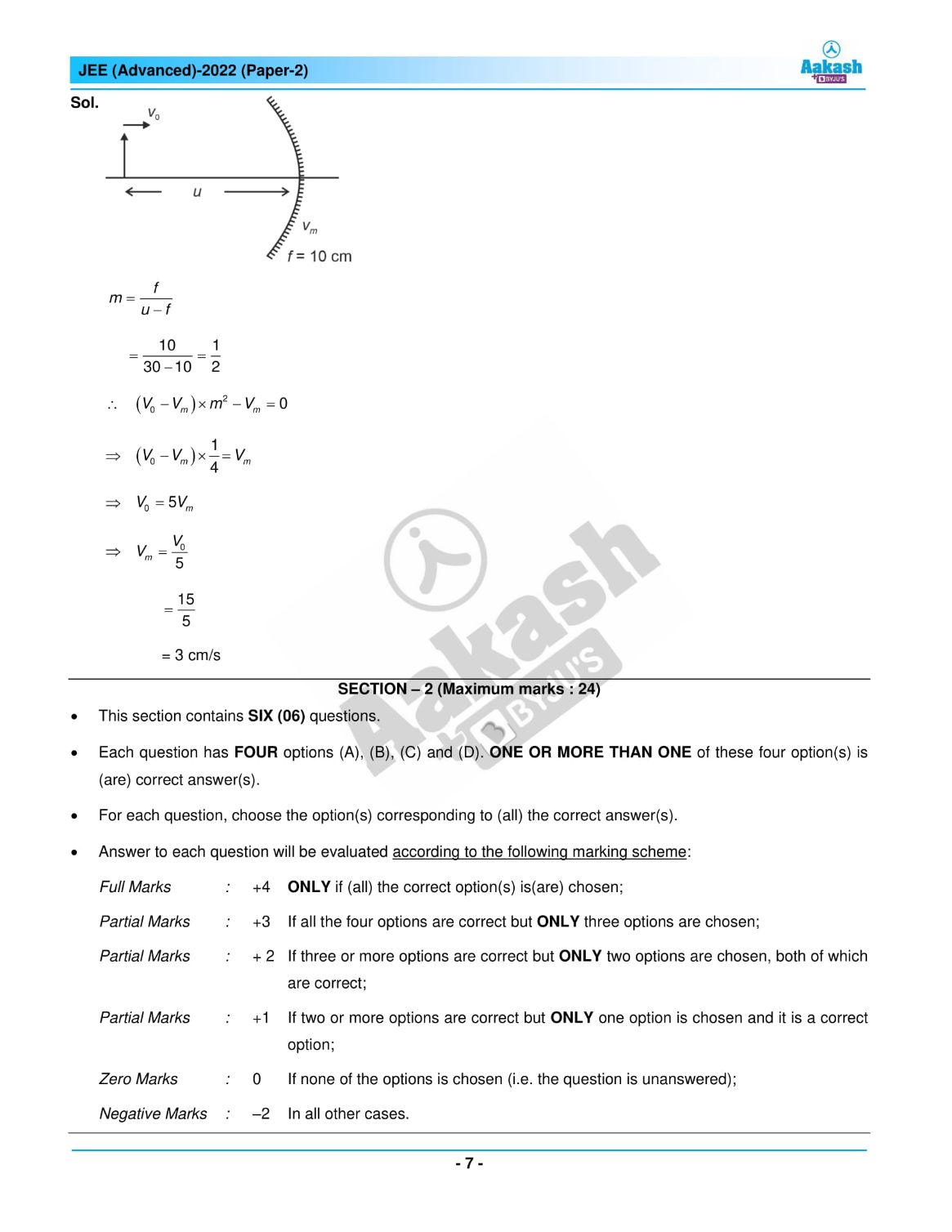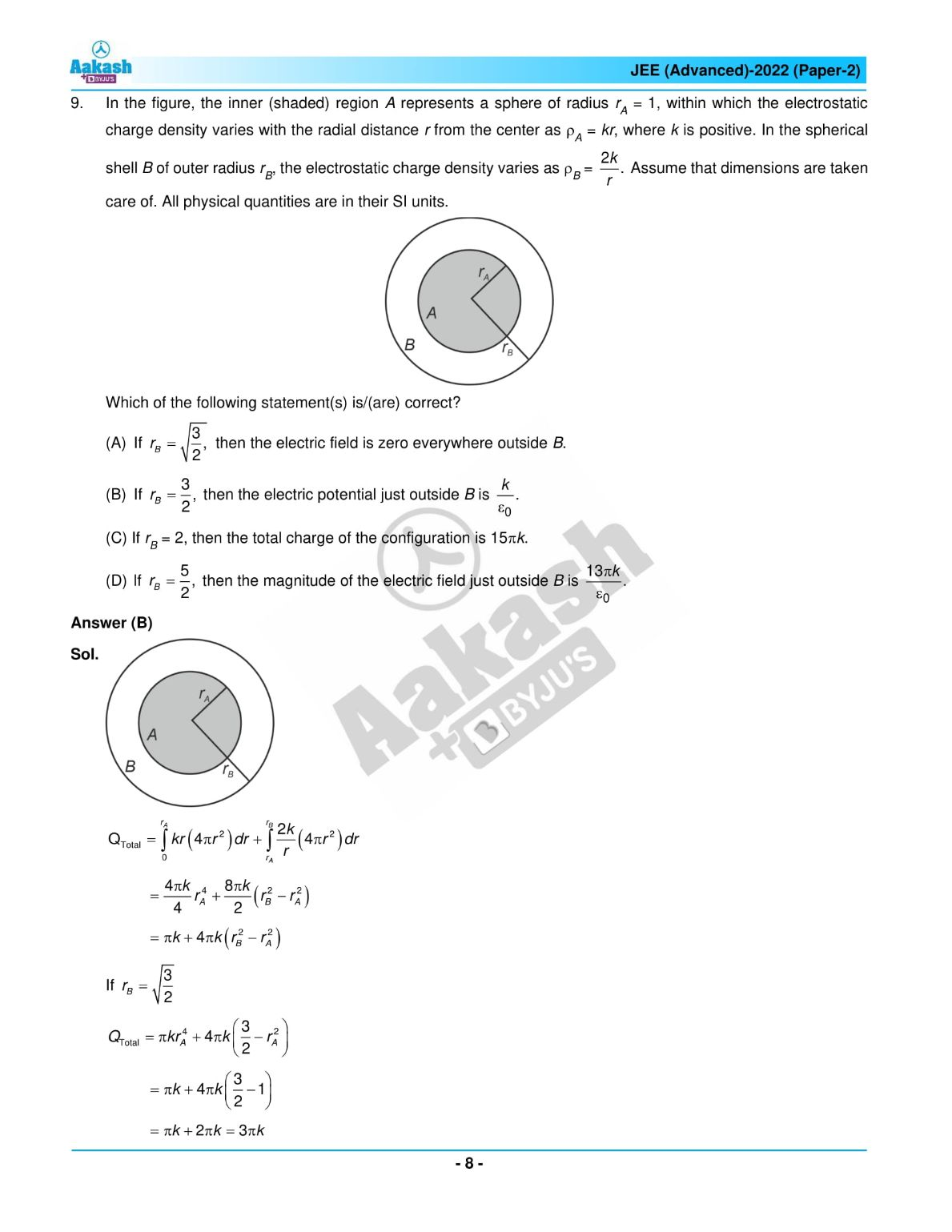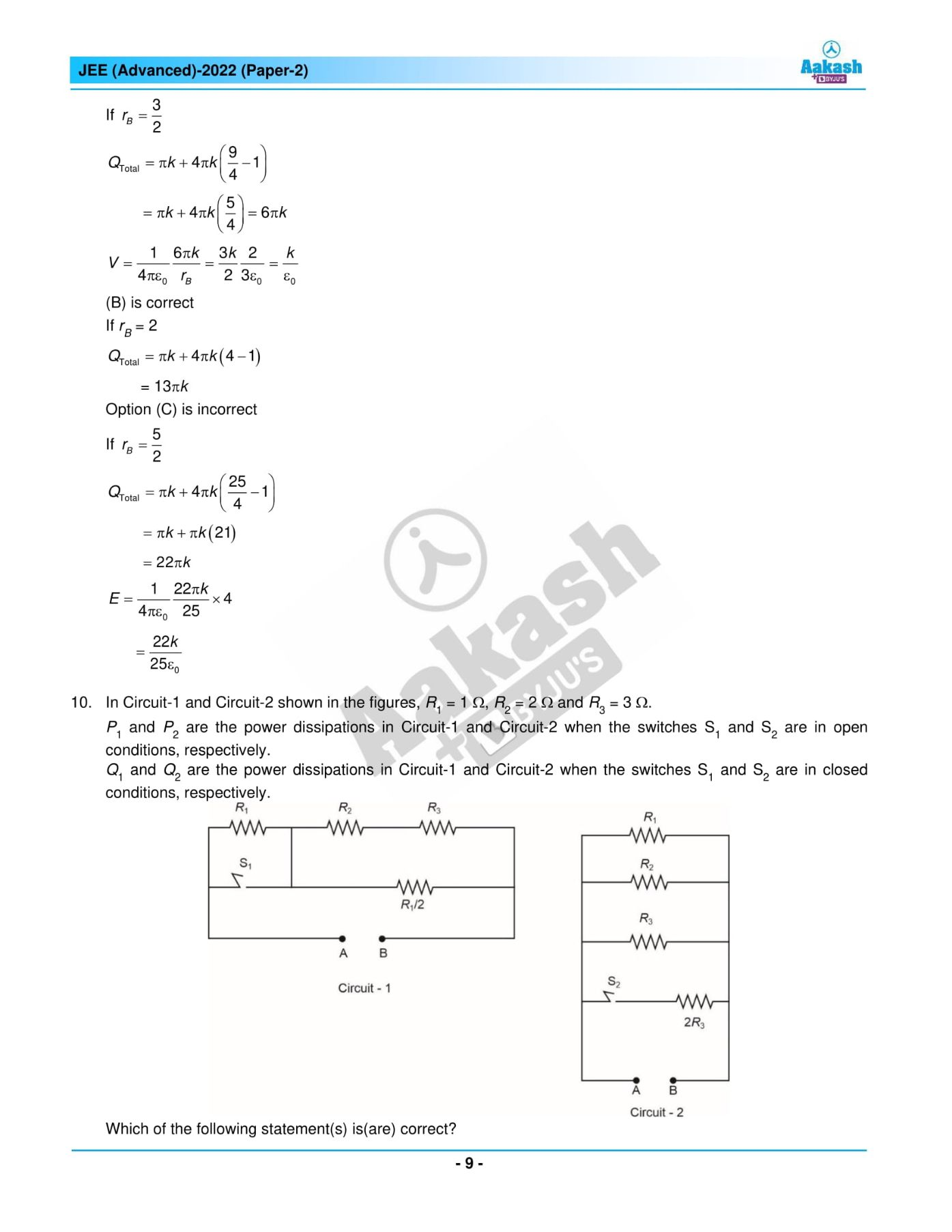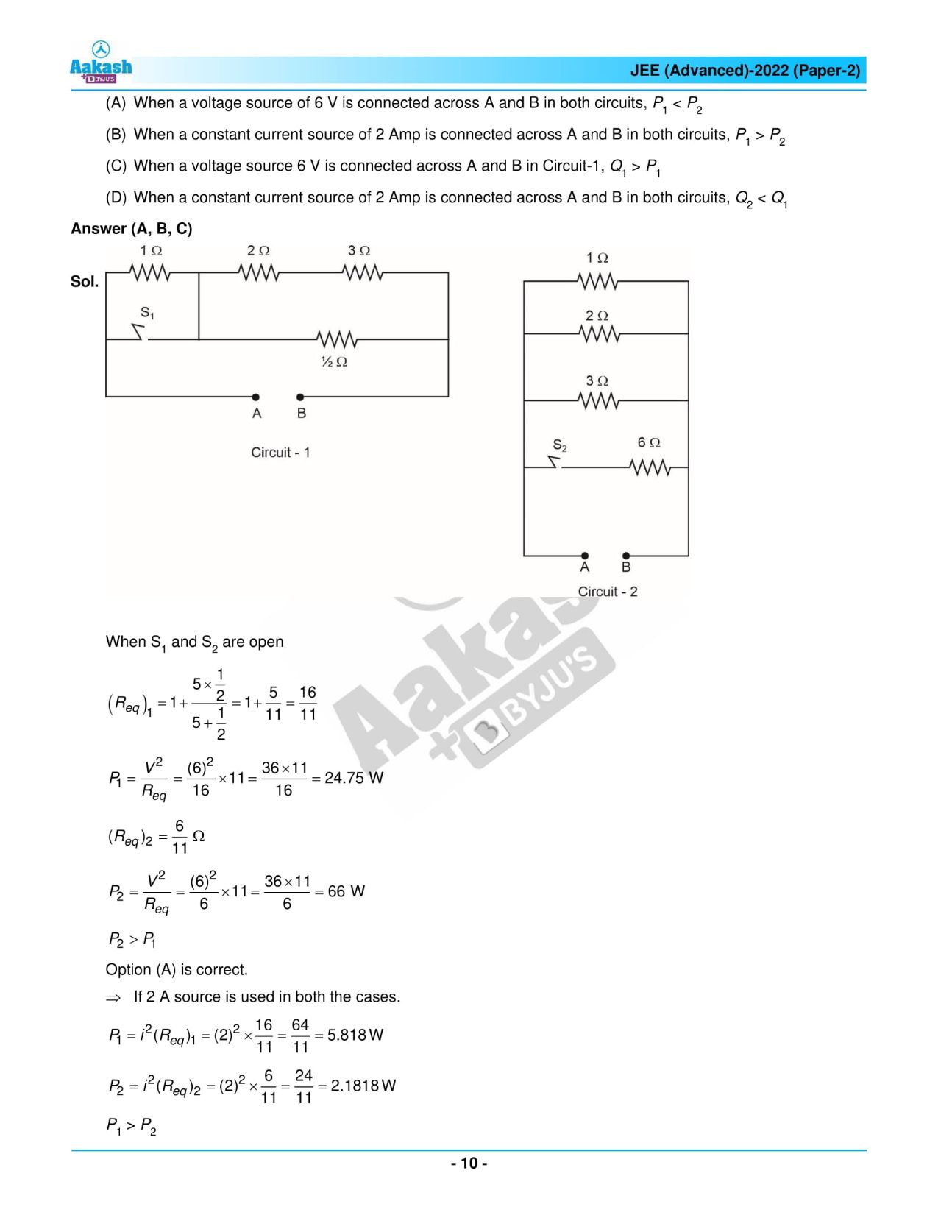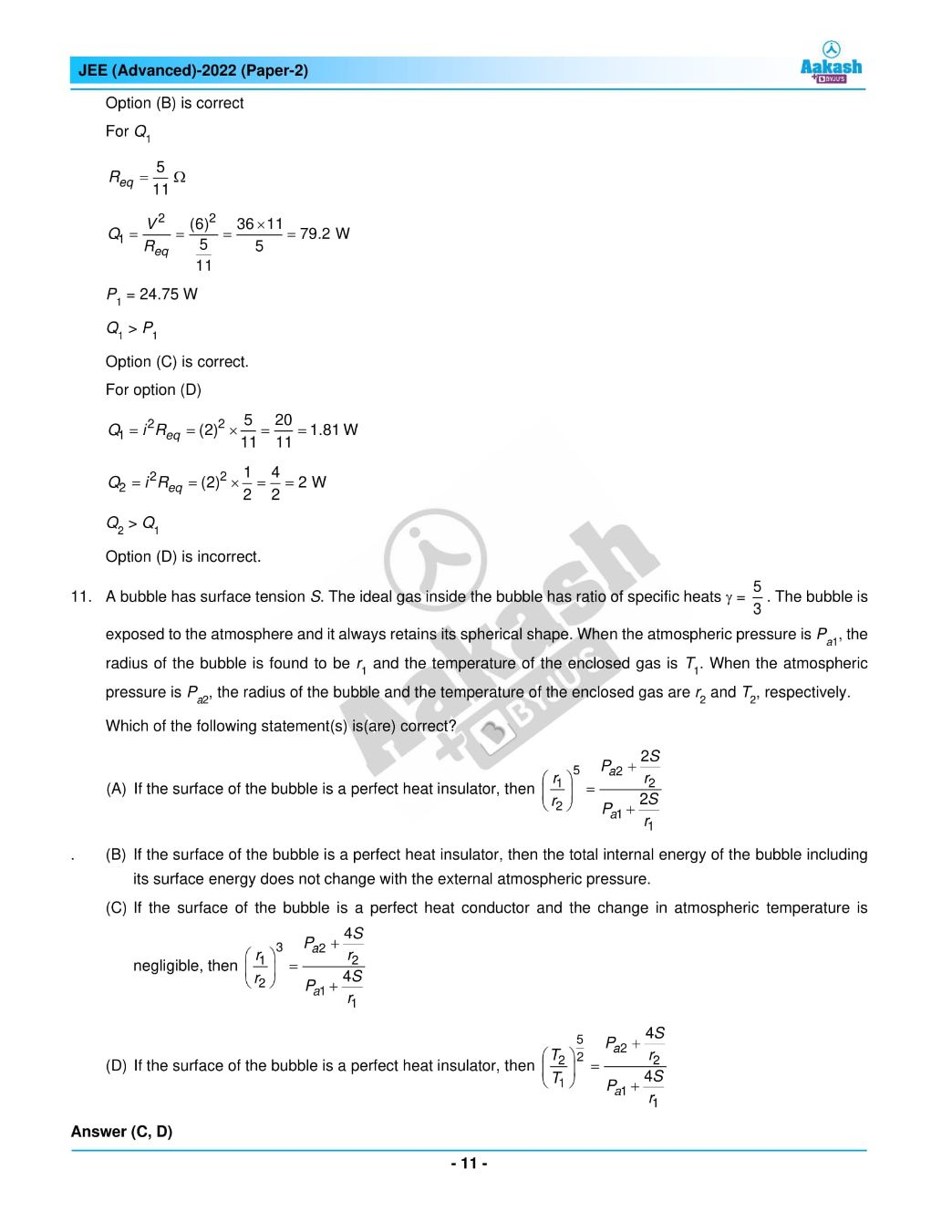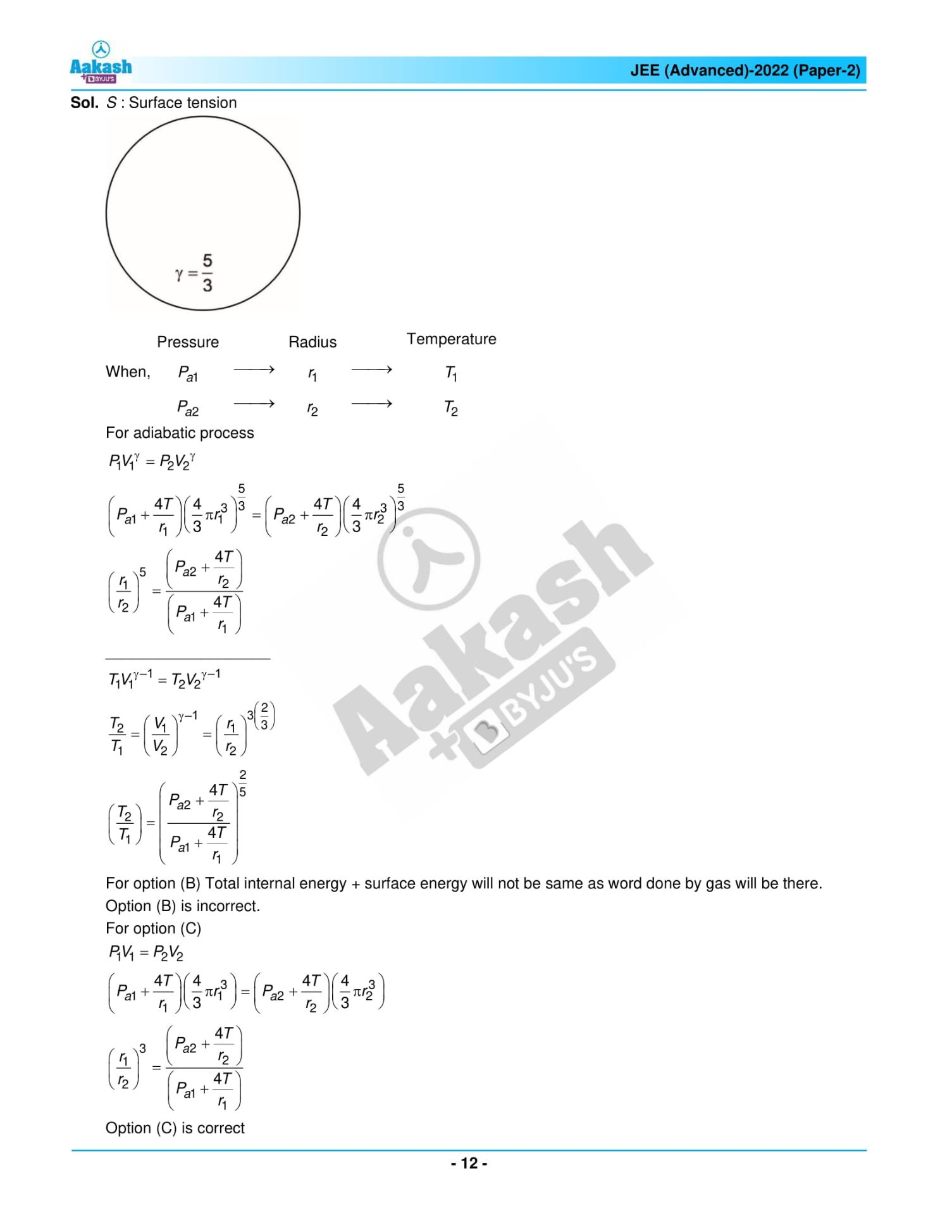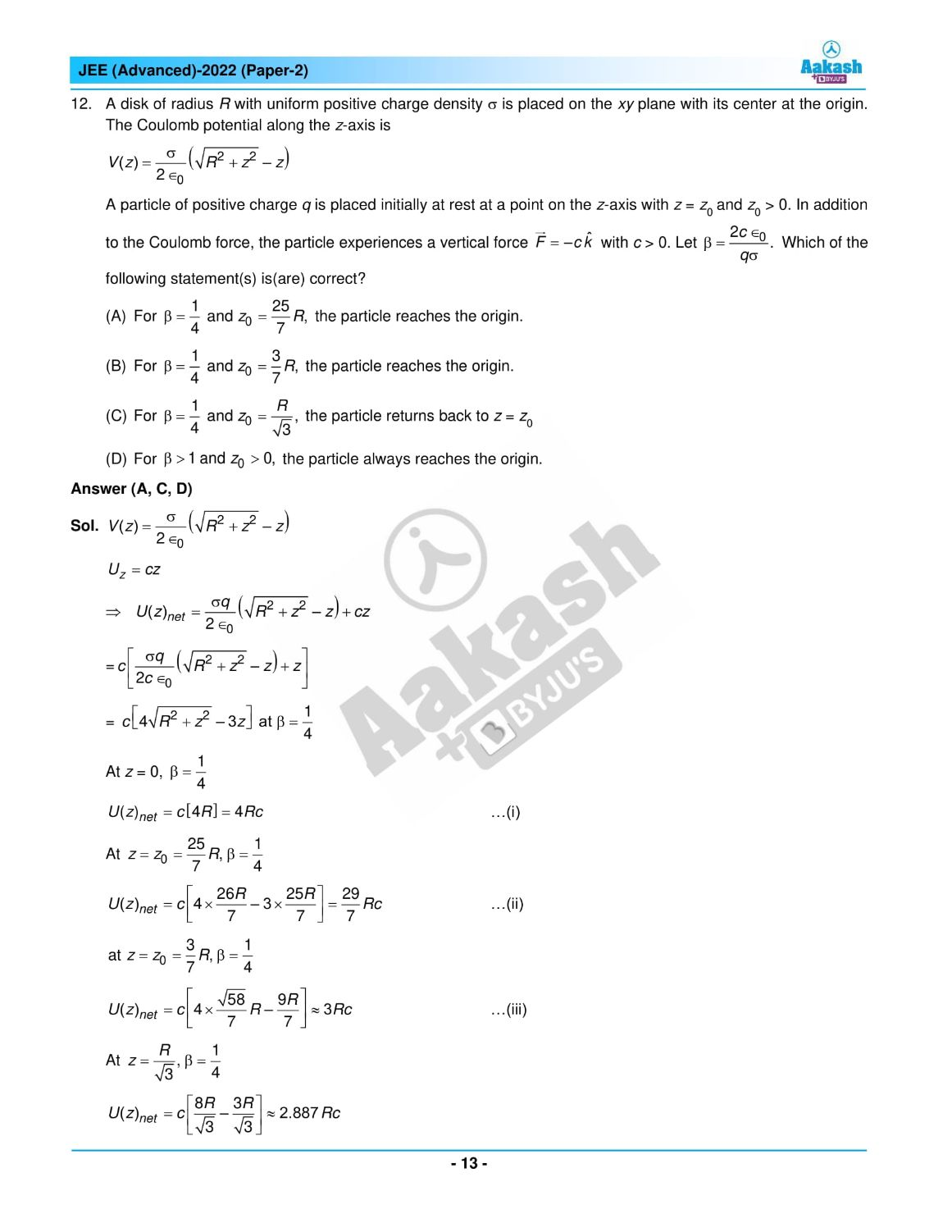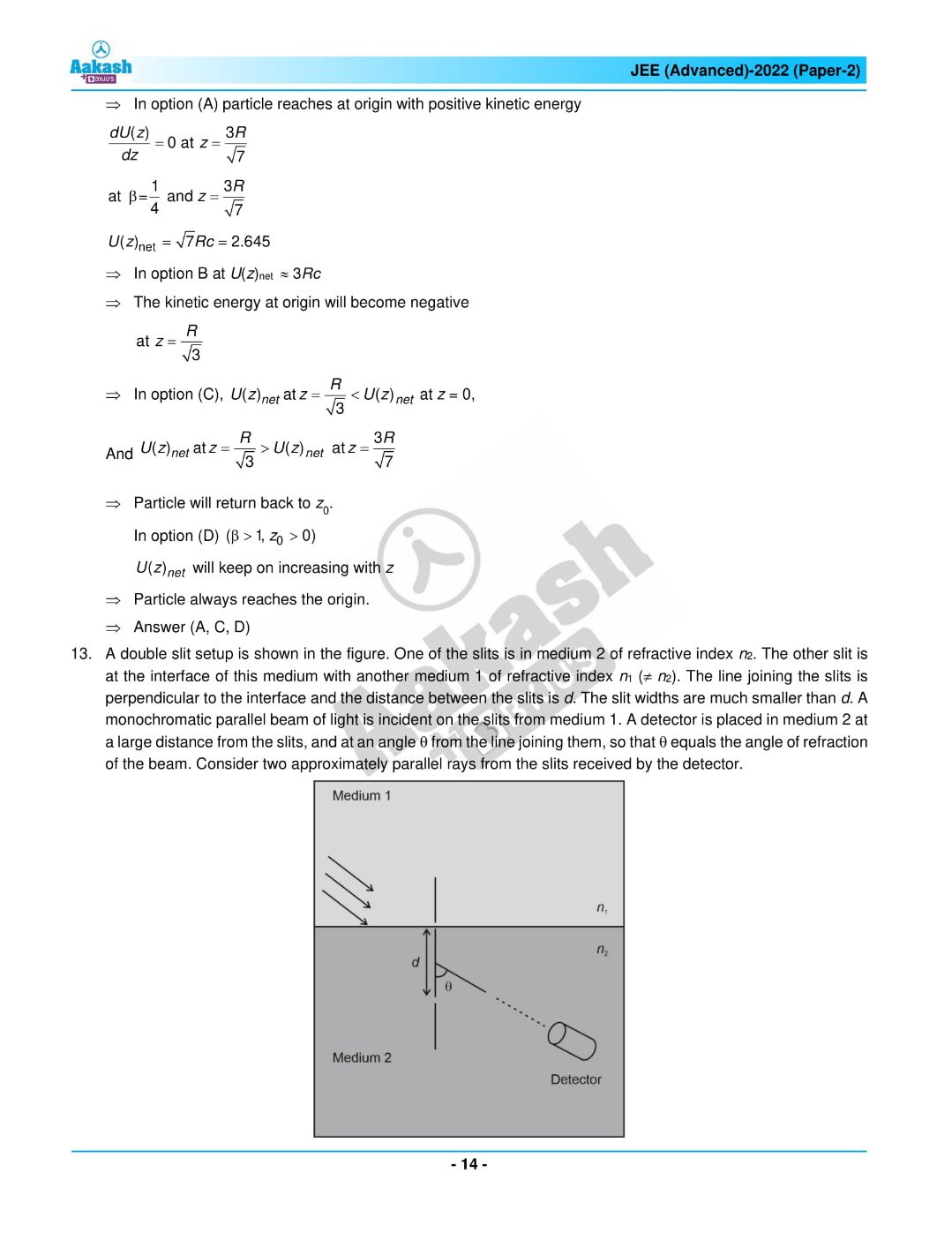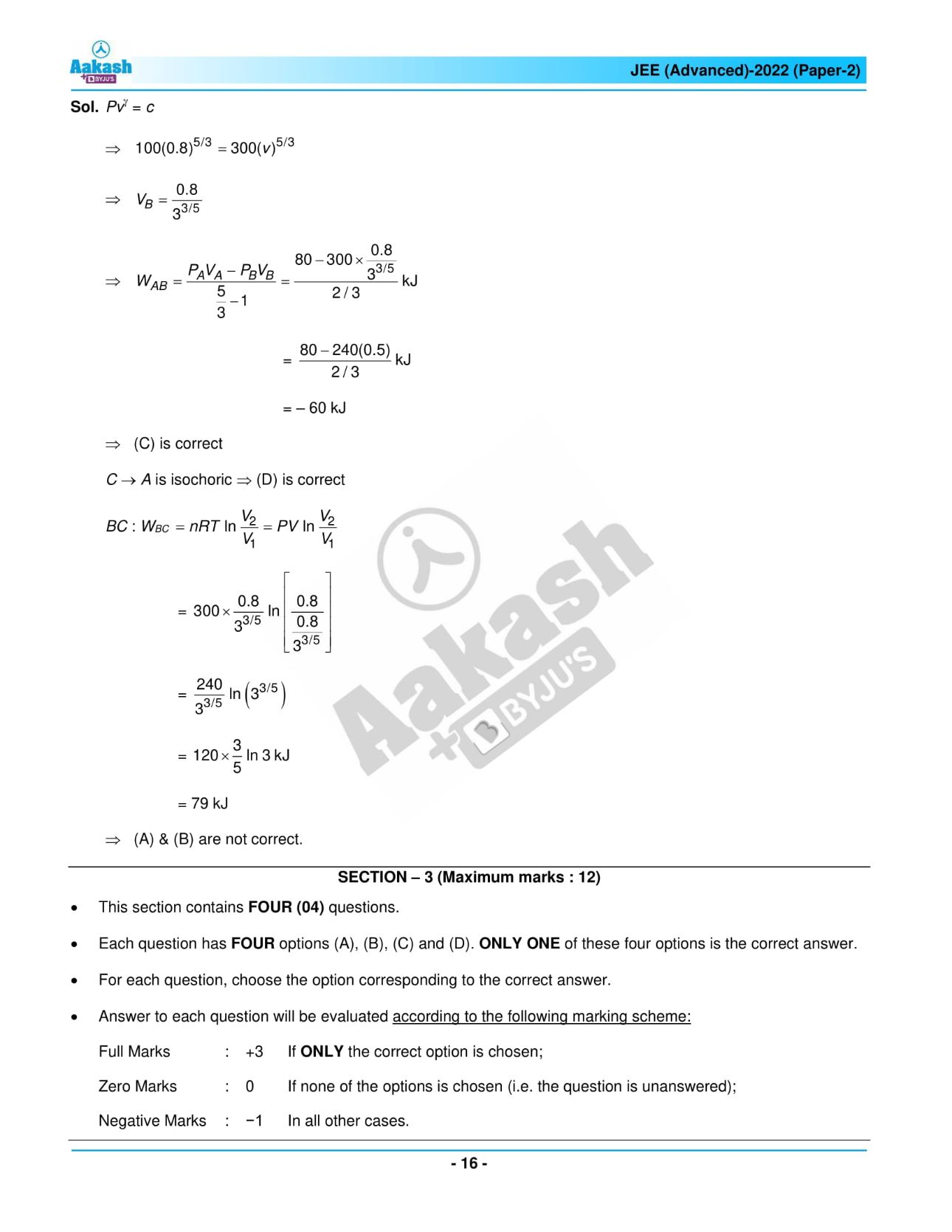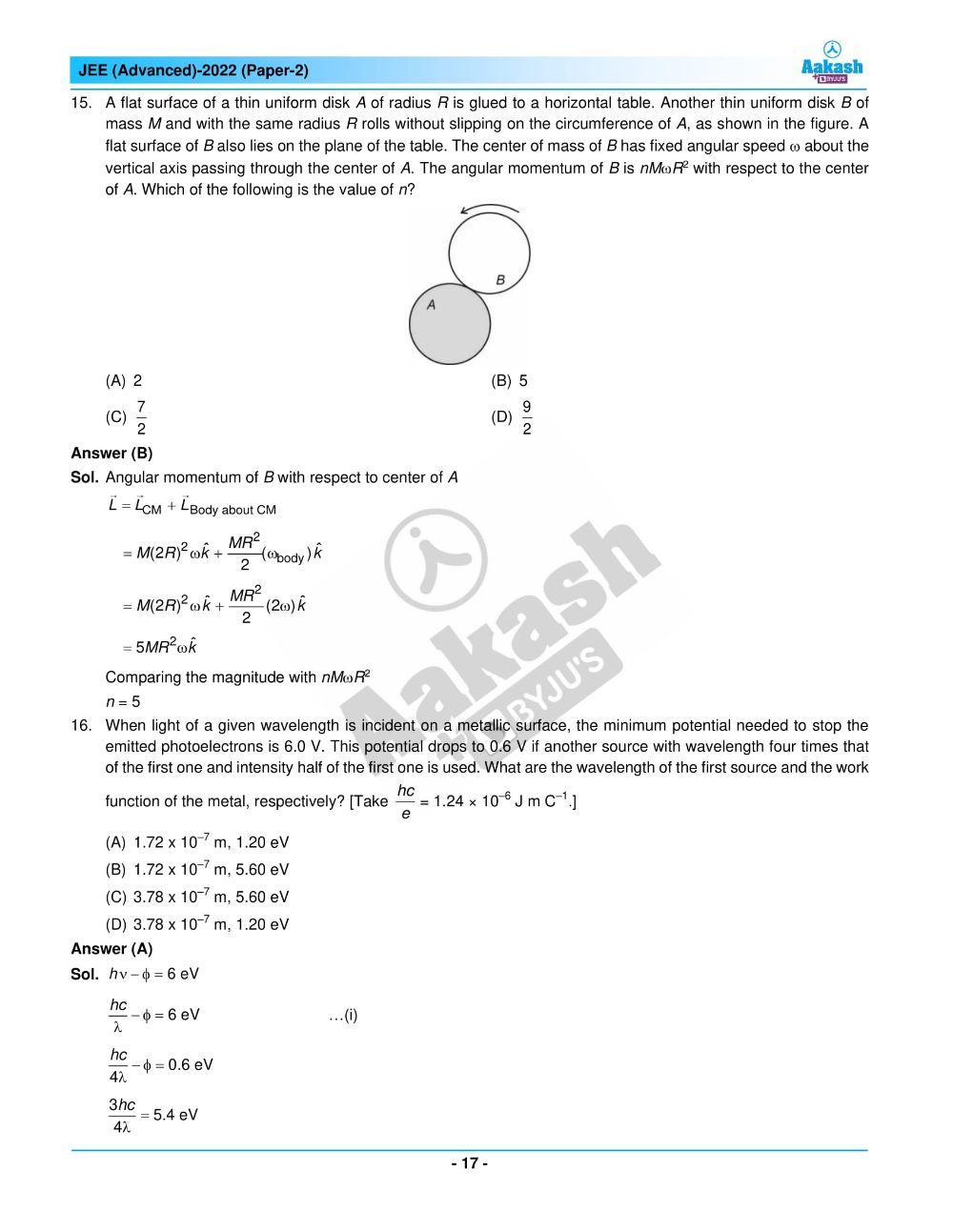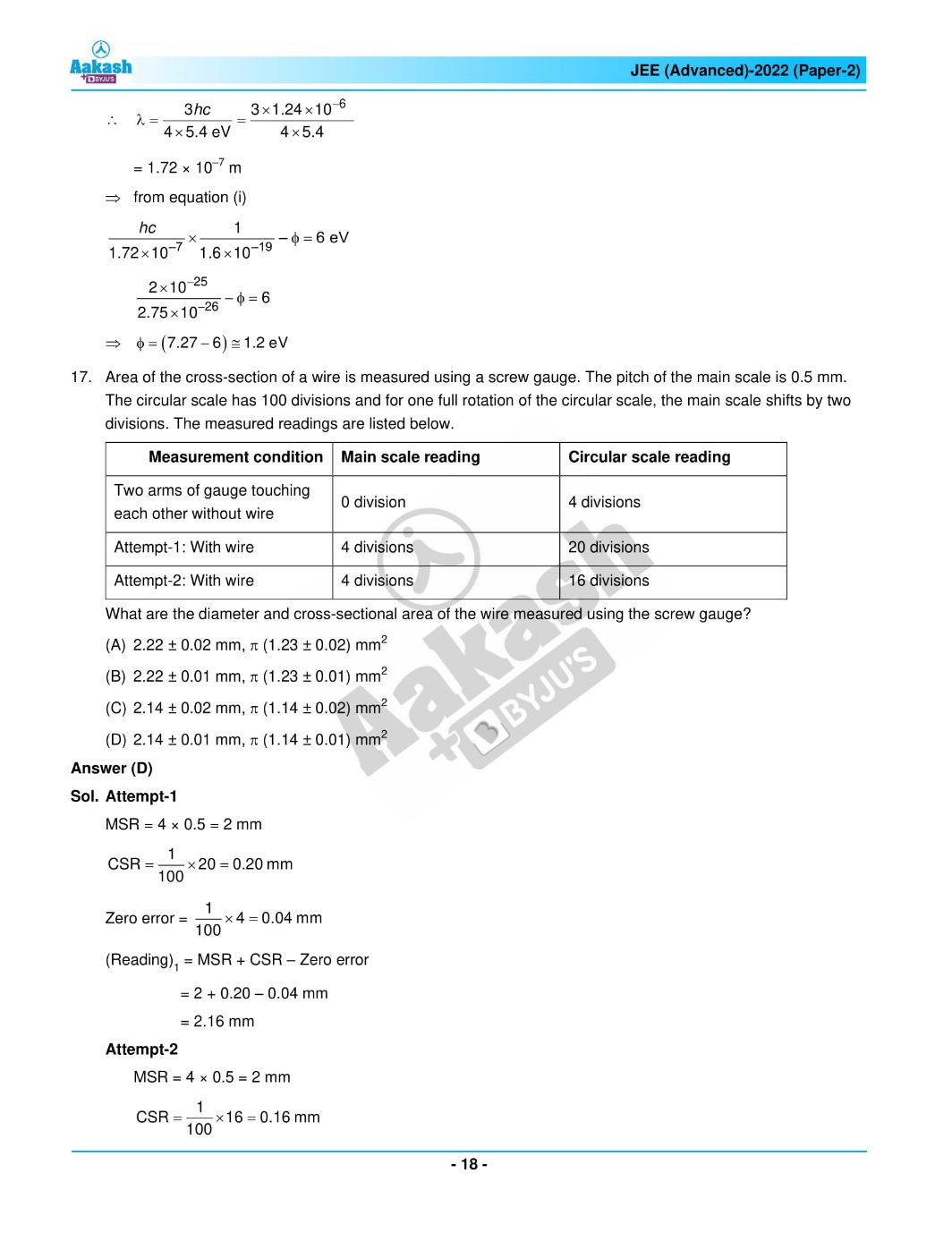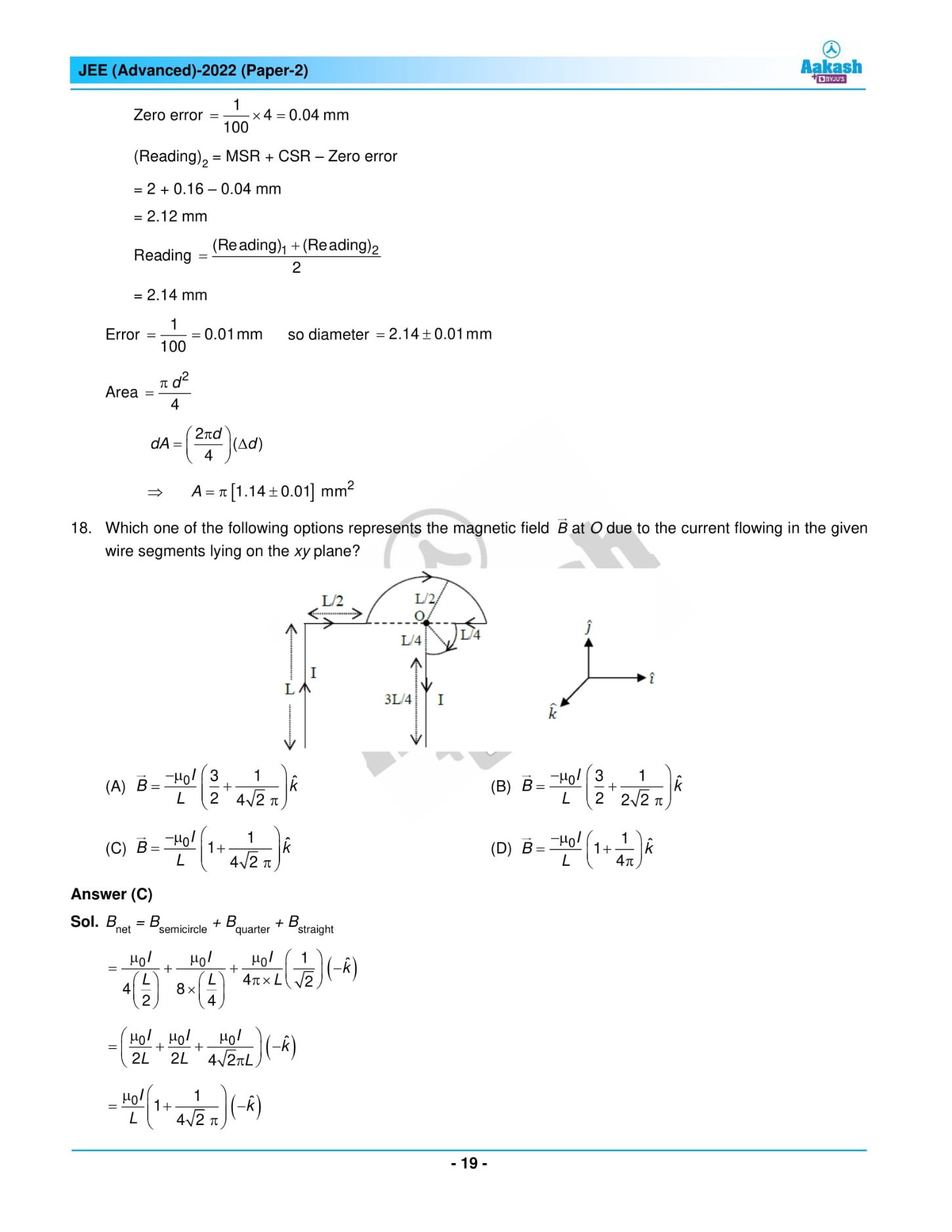## JEE Advanced 2022 Paper 2 – Live Analysis and Solutions## Frequently Asked Questions – FAQs

Q1

### What was the Class-wise distribution of Physics questions in JEE Advanced 2022 Physics Paper 2?

In JEE Advanced 2022 Physics Paper 2, 6 questions were asked from class 11 and 12 from the class 12 syllabus.
Q2

### Was the JEE Advanced 2022 Physics question paper 2 challenging?

The questions asked in the JEE Advanced 2022 Physics question paper 2 were easy to medium to answer.
Q3

### What was the overall difficulty level of Physics Paper 2 in the JEE Advanced 2022 question paper?

The overall difficulty level of Physics Paper 2 in the JEE Advanced 2022 question paper can be stated as 2.11 on a scale of 3.
Q4

### From which chapters are easy questions asked in the JEE Advanced 2022 Physics paper 2?

The chapters from which the easy questions asked in the JEE Advanced 2022 Physics question paper 2 are: Nuclei, System of Particles and Rotational Motion, Dual Nature of Radiation and Matter
Q5

### Is it compulsory to attempt all the questions in the Chemistry part of JEE Advanced 2022 paper 2?

There are three sections in the Chemistry part of JEE Advanced 2022 paper 2, and candidates can attempt the questions based on their choice.